# Category: groups

Here’s a nice, symmetric, labeled graph: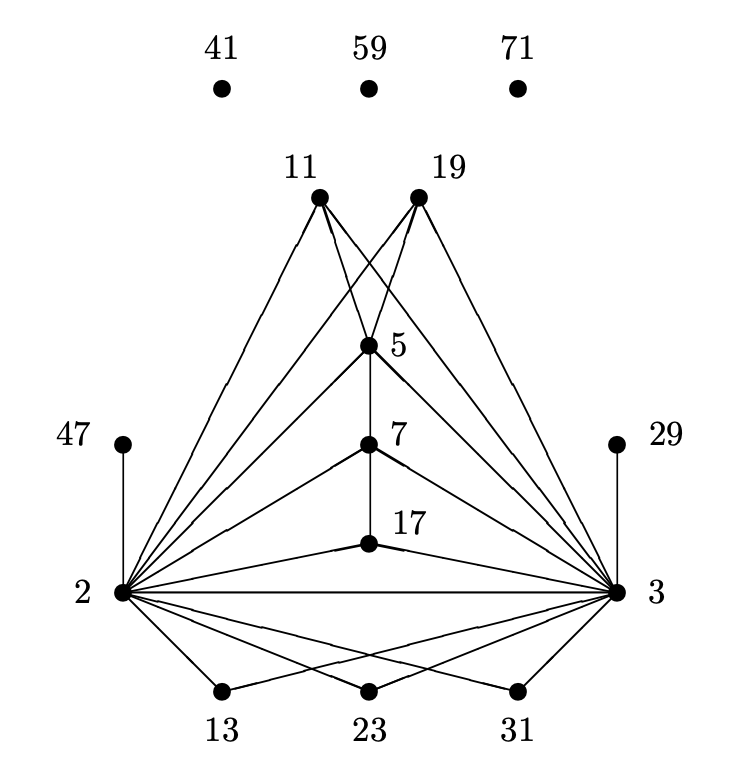The prime numbers labelling the vertices are exactly the prime divisors of the order of the largest sporadic group: the monster group $\mathbb{M}$.
$\# \mathbb{M} = 2^{46}.3^{20}.5^9.7^6.11^2.13^3.17.19.23.29.31.41.47.59.71$

Looking (for example) at the character table of the monster you can check that there is an edge between two primes $p$ and $q$ exactly when the monster has an element of order $p.q$.

Now the fun part: this graph characterises the Monster!

There is no other group $G$ having only elements of these prime orders, and only these edges for its elements of order $p.q$.

This was proved by Melissa Lee and Tomasz Popiel in $\mathbb{M}, \mathbb{B}$, and $\mathbf{Co}_1$ are recognisable by their prime graphs, by using modular character theory.

The proof for the Monster takes less than one page, so it’s clear that it builds on lots of previous results.

There’s the work of Mina Hagie The prime graph of a sporadic simple group, who used the classification of all finite simple groups to put heavy restrictions on possible groups $G$ having the same prime graph as a sporadic simple group.

For the Monster, she proved that if the prime graph of $G$ is that of the monster, then the Fitting subgroup $F(G)$ must be a $3$-group, and $G/F(G) \simeq \mathbb{M}$.

Her result, in turn, builds on the Gruenberg-Kegel theorem, after Karl Gruenberg and Otto Kegel.

The Gruenberg-Kegel theorem, which they never published (a write-up is in the paper Prime graph components of finite groups by Williams), shows the wealth of information contained in the prime graph of a finite group. For this reason, the prime graph is often called the Gruenberg-Kegel graph.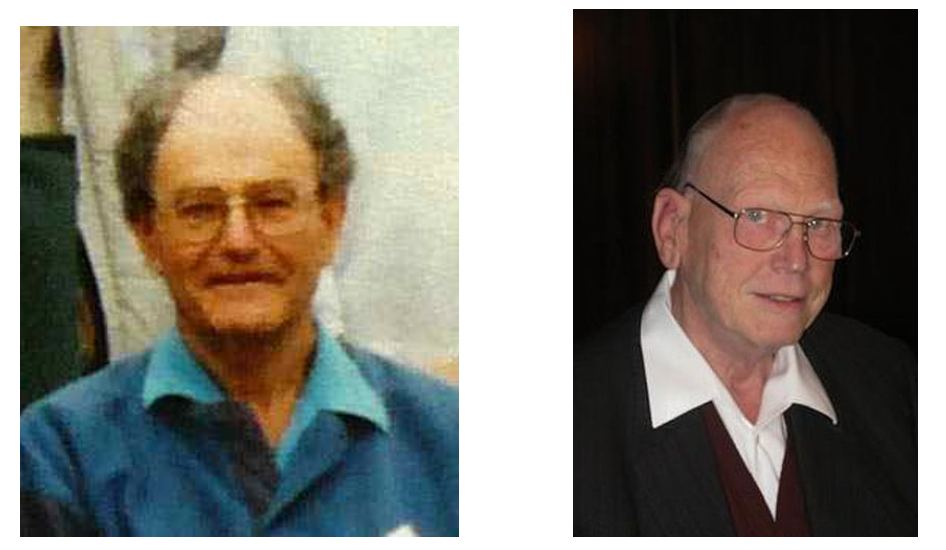The pictures above are taken from a talk by Peter Cameron, The Gruenberg-Kegel graph. Peter Cameron’s blog is an excellent source of information for all things relating groups and graphs.

The full proof of the Gruenberg-Kegel theorem is way too involved for a blogpost, but I should give you at least an idea of it, and of one of the recurrent tools involved, the structural results on Frobenius groups by John Thompson.

Here’s lemma 1.1 of the paper On connection between the structure of a finite group and the properties of its prime graph by A.V. Vasil’ev.

Lemma: If $1 \triangleleft K \triangleleft H \triangleleft G$ is a series of normal subgroups, and if we have primes $p$ dividing the order of $K$, $q$ dividing the order of $H/K$, and $r$ dividing the order of $G/H$, then there is at least one edge among these three vertices in the prime graph of $G$.

Okay, let’s suppose there’s a counterexample $G$, and take one of minimal order. Let $P$ be a Sylow $p$-subgroup of $K$, and $N$ its normaliser in $G$. By the Frattini argument $G=K.N$ and so $G/K \simeq N/(N \cap K)$.

Then there’s a normal series $1 \triangleleft P \triangleleft (N \cap H)=N_H(P) \triangleleft N$, and by Frattini $H=K.(N \cap H)$. But then, $N/(N \cap H)=H.N/H = G/H$ and $(N \cap H)/P$ maps onto $(N \cap H)/(N \cap H \cap K) = H/K$, so this series satisfies the conditions for the three primes $p,q$ and $r$.

But as there is no edge among $p,q$ and $r$ in the prime graph of $G$, there can be no such edge in the prime graph of $N$, and $N$ would be a counterexample of smaller order, unless $N=G$.

Oh, I should have said this before: if there is an edge between two primes in the prime graph of a subgroup (or a quotient) of $G$, then such as edge exists also in the prime group of $G$ (trivial for subgroups, use lifts of elements for quotients).

The only way out is that $N=G$, or that $P$ is a normal subgroup of $G$. Look at quotients $\overline{G}=G/P$ and $\overline{H}=H/P$, take a Sylow $q$-subgroup of $\overline{H}$ and $\overline{M}$ its normaliser in $\overline{G}$.

Frattini again gives $\overline{M}/(\overline{M} \cap \overline{H}) = \overline{G}/\overline{H}$, and $r$ is a prime divisor of the order of $\overline{M}/\overline{Q}$.

Lift the whole schmuck to the lift of $\overline{M}$ in $G$ and get a series of normal subgroups
$1 \triangleleft P \triangleleft Q \triangleleft M$
satisfying the three primes condition, so would give a smaller counter-example unless $M=G$ and $Q$ (the lift of $\overline{Q}$ to $G$) is a normal subgroup of $G$.

Sooner or later, in almost all proofs around the Gruenberg-Kegel result, a Frobenius group enters the picture.

Here, we take an element $x$ in $G$ of order $r$, and consider the subgroup $F$ generated by $Q$ and $x$. The action of $x$ on $Q$ by conjugation is fixed-point free (if not, $G$ would have elements of order $p.r$ or $q.r$ and there is no edge between these prime vertices by assumption).

But then, $F$ is a semi-direct product $Q \rtimes \langle x \rangle$, and again because $G$ has no elements of order $p.r$ nor $q.r$ we have:

• the centraliser-subgroup in $F$ of any non-identity element in $\langle x \rangle$ is contained in $\langle x \rangle$
• the centraliser-subgroup in $F$ of any non-identity element in $Q$ is contained in $Q$

So, $F$ is a Frobenius group with ‘Frobenius kernel’ $Q$. Thompson proved that the Frobenius kernel is a nilpotent group, so a product of its Sylow-subgroups. But then, $Q$ (and therefore $G$) contains an element of order $p.q$, done.

Until now, we’ve looked at actions of groups (such as the $T/I$ or $PLR$-group) or (transformation) monoids (such as Noll’s monoid) on special sets of musical elements, in particular the twelve pitch classes $\mathbb{Z}_{12}$, or the set of all $24$ major and minor chords.

Elephant-lovers recognise such settings as objects in the presheaf topos on the one-object category $\mathbf{M}$ corresponding to the group or monoid. That is, we look at contravariant functors $\mathbf{M} \rightarrow \mathbf{Sets}$.

Last time we’ve encountered the ‘Cube Dance Grap’ which depicts a particular relation among the major, minor, and augmented chords.

Recall that the twelve major chords (numbered for $1$ to $12$) are the ordered triples of tones in $\mathbb{Z}_{12}$ of the form $(n,n+4,n+7)$ (such as the triangle on the left). The twelve minor chords (numbered from $13$ to $24$) are the ordered triples $(n,n+3,n+7)$ (such as the middle triangle). The four augmented chords (numbered from $25$ to $28$) are the triples of the form $(n,n+4,n+8)$ (such as the rightmost triangle).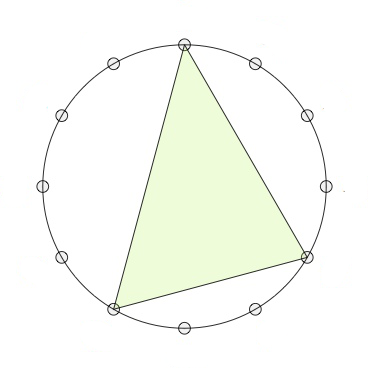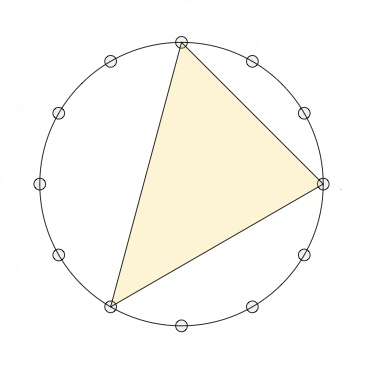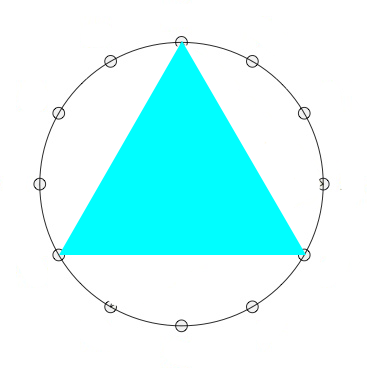The Cube Dance Graph relates two of these chords when they share two tones (pitch classes) whereas the remaining tones differ by a halftone.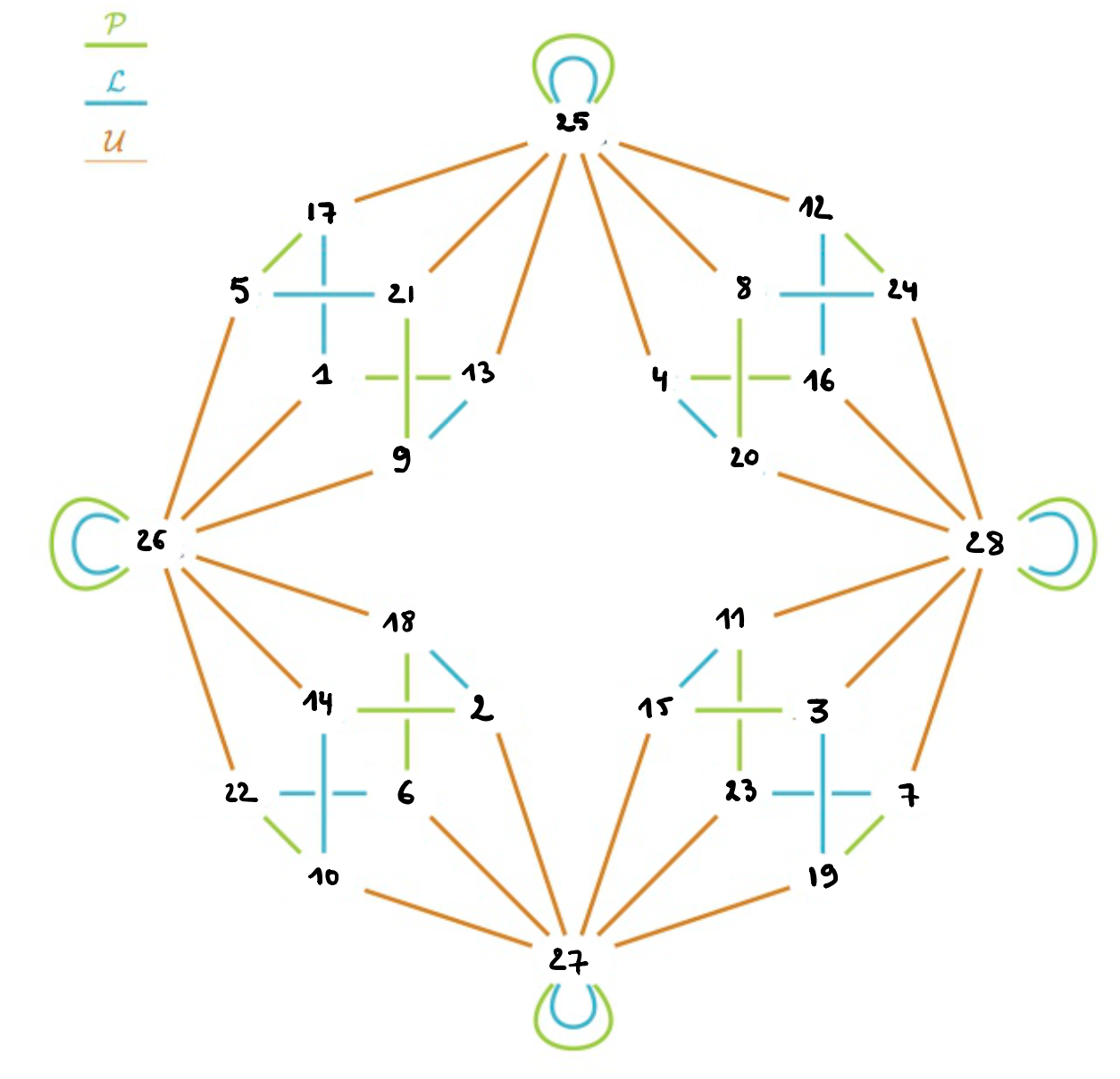Picture modified from this post.

We can separate this symmetric binary relation into three sub-relations: the extension of the $P$ and $L$-operations on major and minor chords to the augmented ones (these are transformations), and the remaining relation $U$ which connects the major and minor chords to the augmented chords (and which is not a transformation).

Binary relations on the same set can be composed, so we get a monoid $\mathbf{M}$ generated by the three relations $P,L$ and $U$. The action of $\mathbf{M}$ on the $28$ chords no longer gives us an ordinary presheaf (because $U$ is not a transformation), but a relational presheaf as in the paper On the use of relational presheaves in transformational music theory by Alexandre Popoff.

That is, the action defines a contravariant functor $\mathbf{M} \rightarrow \mathbf{Rel}$ where $\mathbf{Rel}$ is the category (actually a $2$-category) of sets, but with binary relations as morphisms (that is, $Hom(X,Y)$ is all subsets of $X \times Y$), and the natural notion of composition of such relations. The $2$-morphism between relations is that of inclusion.

To compute with monoids generated by binary relations in GAP one needs to download, compile and load the package semigroups, and to represent the binary relations as partitioned binary relations as in the paper by Martin and Mazorchuk.

This is a bit more complicated than working with ordinary transformations:

 P:=PBR([[-13],[-14],[-15],[-16],[-17],[-18],[-19],[-20],[-21],[-22],[-23],[-24],[-1],[-2],[-3],[-4],[-5],[-6],[-7],[-8],[-9],[-10],[-11],[-12],[-25],[-26],[-27],[-28]],[,,,,,,,,,,,,,,,,,,,,,,,,,,,]); L:=PBR([[-17],[-18],[-19],[-20],[-21],[-22],[-23],[-24],[-13],[-14],[-15],[-16],[-9],[-10],[-11],[-12],[-1],[-2],[-3],[-4],[-5],[-6],[-7],[-8],[-25],[-26],[-27],[-28]],[,,,,,,,,,,,,,,,,,,,,,,,,,,,]); U:=PBR([[-26],[-27],[-28],[-25],[-26],[-27],[-28],[-25],[-26],[-27],[-28],[-25],[-25],[-26],[-27],[-28],[-25],[-26],[-27],[-28],[-25],[-26],[-27],[-28],[-17,-21,-13,-4,-8,-12],[-5,-1,-9,-18,-14,-22],[-2,-6,-10,-15,-23,-19],[-24,-16,-20,-11,-3,-7]],[,,,,,,,,,,,,,,,,,,,,,,,,[17,21,13,4,8,12],[5,1,9,18,14,22],[2,6,10,15,23,19],[24,16,20,11,3,7]]); 

But then, GAP quickly tells us that $\mathbf{M}$ is a monoid consisting of $40$ elements.

 gap> M:=Semigroup([P,L,U]); gap> Size(M); 40 

The Semigroups-package can also compute Green’s relations and tells us that there are seven such $R$-classes, four consisting of $6$ elements, two of four, and one of eight elements. These are also visible in the Cayley graph, exactly as last time.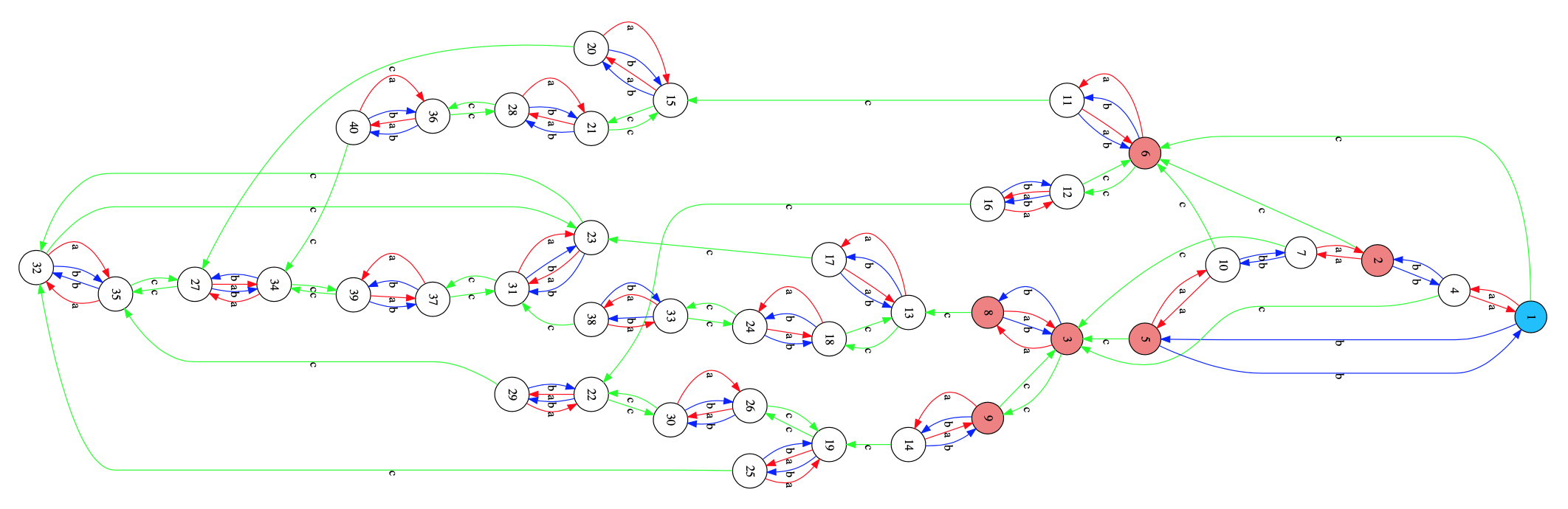Or, if you prefer the cleaner picture of the Cayley graph from the paper Relational poly-Klumpenhouwer networks for transformational and voice-leading analysis by Popoff, Andreatta and Ehresmann.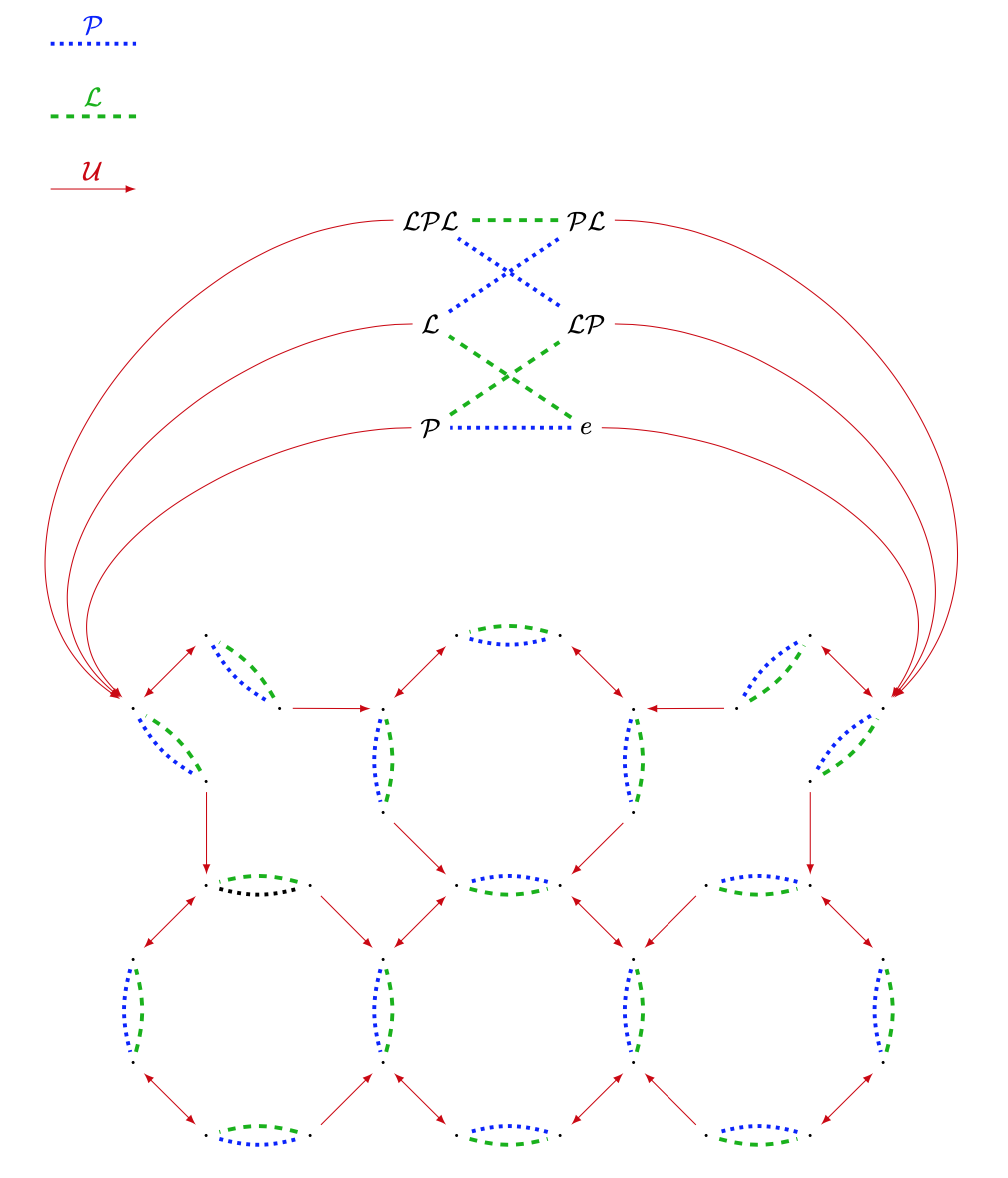This then allows us to compute the Heyting algebra of the subobject classifier, and all the Grothendieck topologies, at least for the ordinary presheaf topos of $\mathbf{M}$-sets, not for the relational presheaves we need here.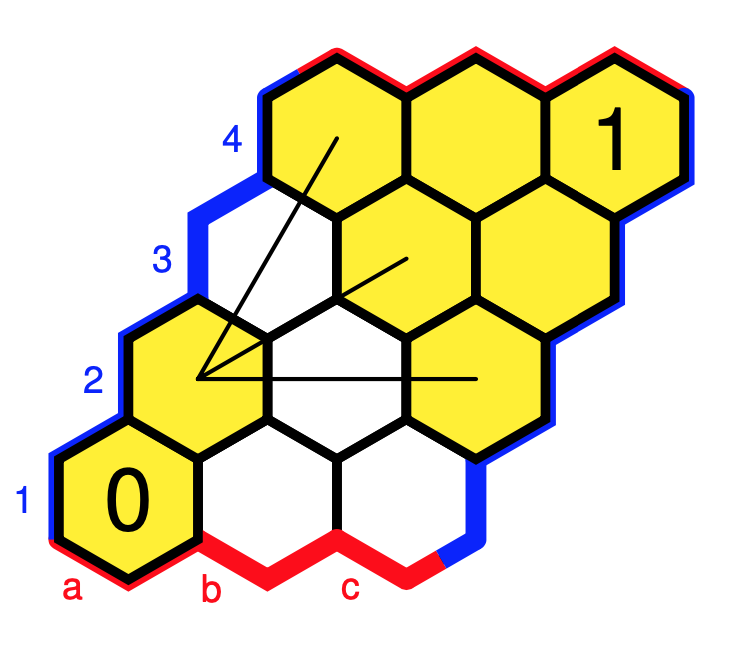We can consider the same binary relation on the larger set of triads when we add the suspended triads. These are the ordered triples in $\mathbb{Z}_{12}$ of the form $(n,n+5,n+7)$, as in the rightmost triangle below.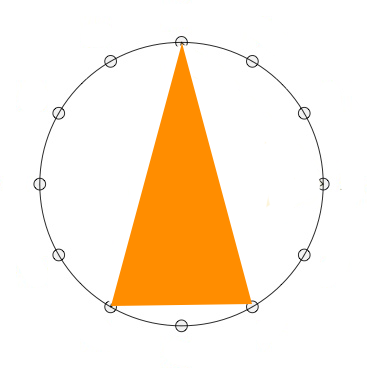There are twelve suspended chords (numbered from $29$ to $40$), so we now have a binary relation $T$ on a set of $40$ triads.

The relation $T$ is too coarse, and the art is to subdivide $T$ is disjoint sub-relations which are musically significant, between major and minor triads, between major/minor and augmented triads, and so on.

For each such partition we can then consider the monoids generated by these sub-relations.

In his paper, Popoff suggest relevant sub-relations $P,L,T_U,T_V$ and $T_U \cup T_V$ of $T$ which in our numbering of the $40$ chords can be represented by these PBR’s (assuming I made no mistakes…ADDED march 24th: I did make a mistake in the definition of L, see comment by Alexandre Popoff, below the corect L):

 P:=PBR([[-13],[-14],[-15],[-16],[-17],[-18],[-19],[-20],[-21],[-22],[-23],[-24],[-1],[-2],[-3],[-4],[-5],[-6],[-7],[-8],[-9],[-10],[-11],[-12],[-25],[-26],[-27],[-28],[-36],[-37],[-38],[-39],[-40],[-29],[-30],[-31],[-32],[-33],[-34],[-35]],[,,,,,,,,,,,,,,,,,,,,,,,,,,,,,,,,,,,,,,,]); L:=PBR([[-17],[-18],[-19],[-20],[-21],[-22],[-23],[-24],[-13],[-14],[-15],[-16],[-9],[ -10],[-11],[-12],[-1],[-2],[-3],[-4],[-5],[-6],[-7],[-8],[-25],[-26],[-27],[-28],[-29], [-30],[-31],[-32],[-33],[-34],[-35],[-36],[-37],[-38],[-39],[-40]],[, , , [ 20],,,,,,,,,,,,,,,,,, , ,,,,,,,,,,,,,,,,, ]); TU:=PBR([[-26],[-27],[-28],[-25],[-26],[-27],[-28],[-25],[-26],[-27],[-28],[-25],[-25],[-26],[-27],[-28],[-25],[-26],[-27],[-28],[-25],[-26],[-27],[-28],[-4,-8,-12,-13,-17,-21],[-1,-5,-9,-14,-18,-22],[-2,-6,-10,-15,-19,-23],[-3,-7,-11,-16,-20,-24],[],[],[],[],[],[],[],[],[],[],[],[]],[,,,,,,,,,,,,,,,,,,,,,,,,[4,8,12,13,17,21],[1,5,9,14,18,22],[2,6,10,15,19,23],[3,7,11,16,20,24],[],[],[],[],[],[],[],[],[],[],[],[]]); TV:=PBR([[-29],[-30],[-31],[-32],[-33],[-34],[-35],[-36],[-37],[-38],[-39],[-40],[-36],[-37],[-38],[-39],[-40],[-29],[-30],[-31],[-32],[-33],[-34],[-35],[],[],[],[],[-1,-18],[-2,-19],[-3,-20],[-4,-21],[-5,-22],[-6,-23],[-7,-24],[-8,-13],[-9,-14],[-10,-15],[-11,-16],[-12,-17]],[,,,,,,,,,,,,,,,,,,,,,,,,[],[],[],[],[1,18],[2,19],[3,20],[4,21],[5,22],[6,23],[7,24],[8,13],[9,14],[10,15],[11,16],[12,17]]); TUV:=PBR([[-26,-29],[-27,-30],[-28,-31],[-25,-32],[-26,-33],[-27,-34],[-28,-35],[-25,-36],[-26,-37],[-27,-38],[-28,-39],[-25,-40],[-25,-36],[-26,-37],[-27,-38],[-28,-39],[-25,-40],[-26,-29],[-27,-30],[-28,-31],[-25,-32],[-26,-33],[-27,-34],[-28,-35],[-4,-8,-12,-13,-17,-21],[-1,-5,-9,-14,-18,-22],[-2,-6,-10,-15,-19,-23],[-3,-7,-11,-16,-20,-24],[-1,-18],[-2,-19],[-3,-20],[-4,-21],[-5,-22],[-6,-23],[-7,-24],[-8,-13],[-9,-14],[-10,-15],[-11,-16],[-12,-17]],[[26,29],[27,30],[28,31],[25,32],[26,33],[27,34],[28,35],[25,36],[26,37],[27,38],[28,39],[25,40],[25,36],[26,37],[27,38],[28,39],[25,40],[26,29],[27,30],[28,31],[25,32],[26,33],[27,34],[28,35],[4,8,12,13,17,21],[1,5,9,14,18,22],[2,6,10,15,19,23],[3,7,11,16,20,24],[1,18],[2,19],[3,20],[4,21],[5,22],[6,23],[7,24],[8,13],[9,14],[10,15],[11,16],[12,17]]); 

The resulting monoids are huge:

 gap> G:=Semigroup([P,L,TU,TV]); gap> Size(G); 473293 gap> H:=Semigroup([P,L,TUV]); gap> Size(H); 994624 

In Popoff’s paper these monoids have sizes respectively $473,293$ and $994,624$. Strangely, the offset is in both cases $144=12^2$. (Added march 24: with the correct L I get the same sizes as in Popoff’s paper).

Perhaps we should try to transform such relational presheaves to ordinary presheaves.

One approach is to use the Grothendieck construction and associate to a set with such a relational monoid action a directed graph, coloured by the elements of the monoid. That is, an object in the presheaf topos of the category
$\xymatrix{C & E \ar[l]^c \ar@/^2ex/[r]^s \ar@/_2ex/[r]_t & V}$
and then we should consider the slice topos over the one-vertex bouquet graph with one loop for each element in the monoid.

If you want to have more details on the musical side of things, for example if you want to know what the opening twelve chords of “Take a Bow” by Muse have to do with the Cube Dance graph, here are some more papers:

A categorical generalization of Klumpenhouwer networks, A. Popoff, M. Andreatta and A. Ehresmann.

From K-nets to PK-nets: a categorical approach, A. Popoff, M. Andreatta and A. Ehresmann.

From a Categorical Point of View: K-Nets as Limit Denotators, G. Mazzola and M. Andreatta.

Last time, we’ve viewed major and minor triads (chords) as inscribed triangles in a regular $12$-gon.If we move clockwise along the $12$-gon, starting from the endpoint of the longest edge (the root of the chord, here the $0$-vertex) the edges skip $3,2$ and $4$ vertices (for a major chord, here on the left the major $0$-chord) or $2,3$ and $4$ vertices (for a minor chord, here on the right the minor $0$-chord).

The symmetries of the $12$-gon, the dihedral group $D_{12}$, act on the $24$ major- and minor-chords transitively, preserving the type for rotations, and interchanging majors with minors for reflections.

Mathematical Music Theoreticians (MaMuTh-ers for short) call this the $T/I$-group, and view the rotations of the $12$-gon as transpositions $T_k : x \mapsto x+k~\text{mod}~12$, and the reflections as involutions $I_k : x \mapsto -x+k~\text{mod}~12$.

Note that the elements of the $T/I$-group act on the vertices of the $12$-gon, from which the action on the chord-triangles follows.

There is another action on the $24$ major and minor chords, mapping a chord-triangle to its image under a reflection in one of its three sides.

Note that in this case the reflection $I_k$ used will depend on the root of the chord, so this action on the chords does not come from an action on the vertices of the $12$-gon.

There are three such operations: (pictures are taken from Alexandre Popoff’s blog, with the ‘funny names’ removed)

The $P$-operation is reflection in the longest side of the chord-triangle. As the longest side is preserved, $P$ interchanges the major and minor chord with the same root.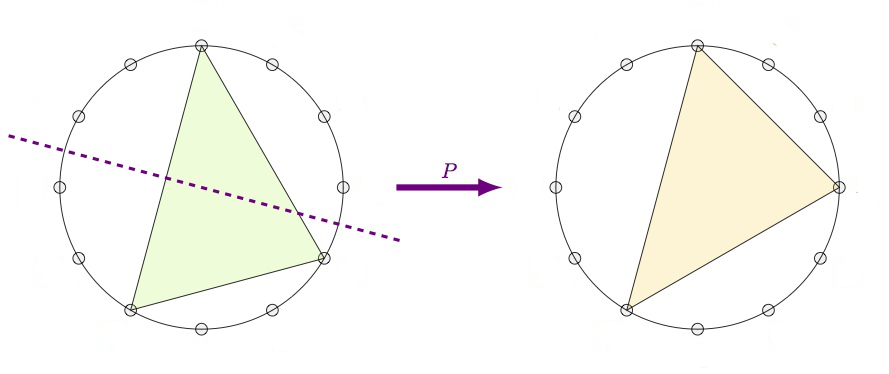The $L$-operation is refection in the shortest side. This operation interchanges a major $k$-chord with a minor $k+4~\text{mod}~12$-chord.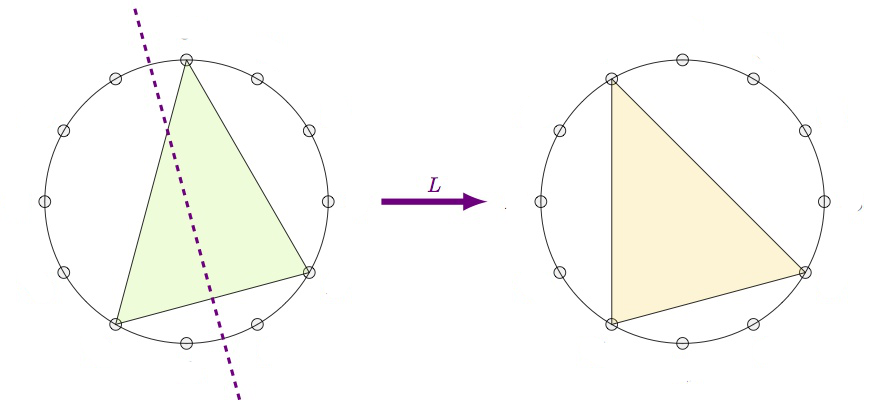Finally, the $R$-operation is reflection in the middle side. This operation interchanges a major $k$-chord with a minor $k+9~\text{mod}~12$-chord.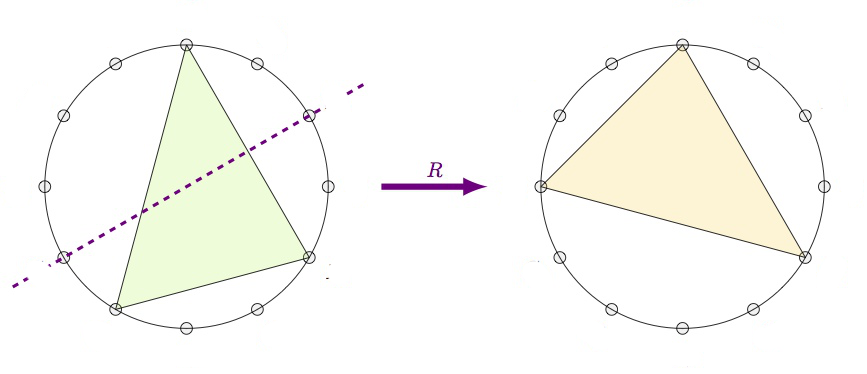From this it is already clear that the group generated by $P$, $L$ and $R$ acts transitively on the $24$ major and minor chords, but what is this $PLR$-group?

If we label the major chords by their root-vertex $1,2,\dots,12$ (GAP doesn’t like zeroes), and the corresponding minor chords $13,14,\dots,24$, then these operations give these permutations on the $24$ chords:

 P:=(1,13)(2,14)(3,15)(4,16)(5,17)(6,18)(7,19)(8,20)(9,21)(10,22)(11,23)(12,24) L:=(1,17)(2,18)(3,19)(4,20)(5,21)(6,22)(7,23)(8,24)(9,13)(10,14)(11,15)(12,16) R:=(1,22)(2,23)(3,24)(4,13)(5,14)(6,15)(7,16)(8,17)(9,18)(10,19)(11,20)(12,21) 

Then GAP gives us that the $PLR$-group is again isomorphic to $D_{12}$:

 gap> G:=Group(P,L,R);; gap> Size(G); 24 gap> IsDihedralGroup(G); true 

In fact, if we view both the $T/I$-group and the $PLR$-group as subgroups of the symmetric group $Sym(24)$ via their actions on the $24$ major and minor chords, these groups are each other centralizers! That is, the $T/I$-group and $PLR$-group are dual to each other.

For more on this, there’s a beautiful paper by Alissa Crans, Thomas Fiore and Ramon Satyendra: Musical Actions of Dihedral Groups.

What does this new MaMuTh info learns us more about our Elephant, the Topos of Triads, studied by Thomas Noll?

Last time we’ve seen the eight element triadic monoid $T$ of all affine maps preserving the three tones $\{ 0,4,7 \}$ of the major $0$-chord, computed the subobject classified $\Omega$ of the corresponding topos of presheaves, and determined all its six Grothendieck topologies, among which were these three: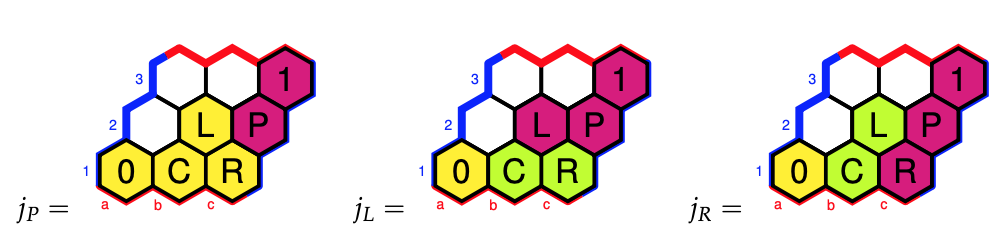Why did we label these Grothendieck topologies (and corresponding elements of $\Omega$) by $P$, $L$ and $R$?

We’ve seen that the sheafification of the presheaf $\{ 0,4,7 \}$ in the triadic topos under the Grothendieck topology $j_P$ gave us the sheaf $\{ 0,3,4,7 \}$, and these are the tones of the major $0$-chord together with those of the minor $0$-chord, that is the two chords in the $\langle P \rangle$-orbit of the major $0$-chord. The group $\langle P \rangle$ is the cyclic group $C_2$.

For the sheafication with respect to $j_L$ we found the $T$-set $\{ 0,3,4,7,8,11 \}$ which are the tones of the major and minor $0$-,$4$-, and $8$-chords. Again, these are exactly the six chords in the $\langle P,L \rangle$-orbit of the major $0$-chord. The group $\langle P,L \rangle$ is isomorphic to $Sym(3)$.

The $j_R$-topology gave us the $T$-set $\{ 0,1,3,4,6,7,9,10 \}$ which are the tones of the major and minor $0$-,$3$-, $6$-, and $9$-chords, and lo and behold, these are the eight chords in the $\langle P,R \rangle$-orbit of the major $0$-chord. The group $\langle P,R \rangle$ is the dihedral group $D_4$.

More on this can be found in the paper Commuting Groups and the Topos of Triads by Thomas Fiore and Thomas Noll.

The operations $P$, $L$ and $R$ on major and minor chords are reflexions in one side of the chord-triangle, so they preserve two of the three tones. There’s a distinction between the $P$ and $L$ operations and $R$ when it comes to how the third tone changes.

Under $P$ and $L$ the third tone changes by one halftone (because the corresponding sides skip an even number of vertices), whereas under $R$ the third tone changes by two halftones (a full tone), see the pictures above.

The $\langle P,L \rangle = Sym(3)$ subgroup divides the $24$ chords in four orbits of six chords each, three major chords and their corresponding minor chords. These orbits consist of the

• $0$-, $4$-, and $8$-chords (see before)
• $1$-, $5$-, and $9$-chords
• $2$-, $6$-, and $10$-chords
• $3$-, $7$-, and $11$-chords

and we can view each of these orbits as a cycle tracing six of the eight vertices of a cube with one pair of antipodal points removed.

These four ‘almost’ cubes are the NE-, SE-, SW-, and NW-regions of the Cube Dance Graph, from the paper Parsimonious Graphs by Jack Douthett and Peter Steinbach.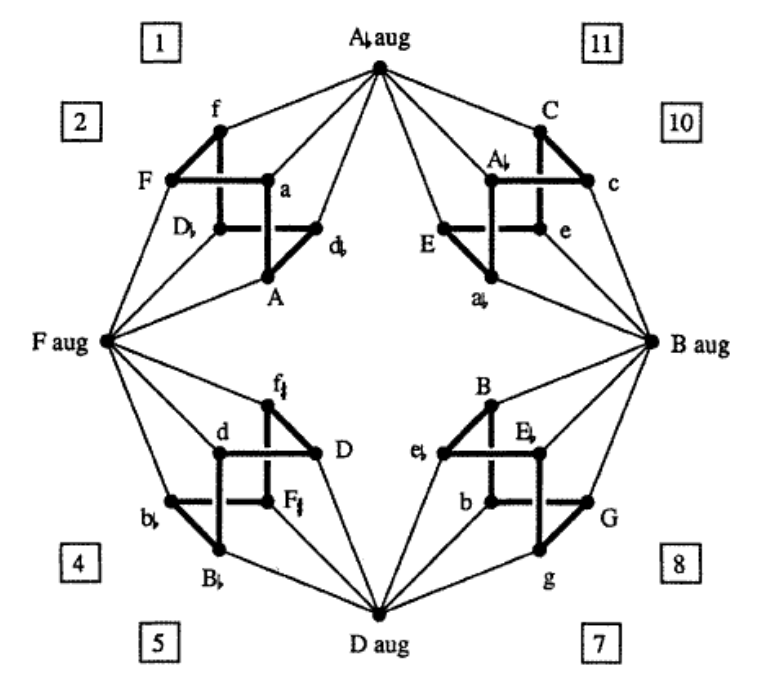To translate the funny names to our numbers, use this dictionary (major chords are given by a capital letter):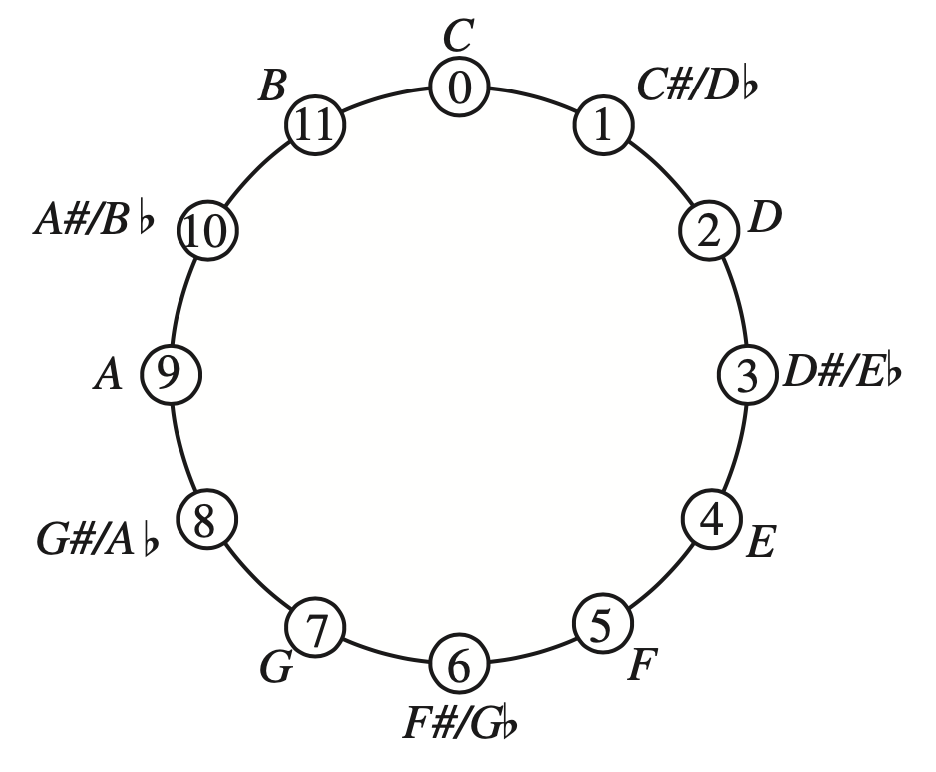The four extra chords (at the N, E, S, and P places) are augmented triads. They correspond to the triads $(0,4,8),~(1,5,9),~(2,6,10)$ and $(3,7,11)$.

That is, two triads are connected by an edge in the Cube Dance graph if they share two tones and differ by an halftone in the third tone.

This graph screams for a group or monoid acting on it. Some of the edges we’ve already identified as the action of $P$ and $L$ on the $24$ major and minor triads. Because the triangle of an augmented triad is equilateral, we see that they are preserved under $P$ and $L$.

But what about the edges connecting the regular triads to the augmented ones? If we view each edge as two directed arrows assigned to the same operation, we cannot do this with a transformation because the operation sends each augmented triad to six regular triads.

Alexandre Popoff, Moreno Andreatta and Andree Ehresmann suggest in their paper Relational poly-Klumpenhouwer networks for transformational and voice-leading analysis that one might use a monoid generated by relations, and they show that there is such a monoid with $40$ elements acting on the Cube Dance graph.

Popoff claims that usual presheaf toposes, that is contravariant functors to $\mathbf{Sets}$ are not enough to study transformational music theory. He suggest to use instead functors to $\mathbf{Rel}$, that is Sets with as the morphisms binary relations, and their compositions.

Another Elephant enters the room…

(to be continued)

Here, MaMuTh stands for Mathematical Music Theory which analyses the pitch, timing, and structure of works of music.

The Elephant is the nickname for the ‘bible’ of topos theory, Sketches of an Elephant: A Topos Theory Compendium, a two (three?) volume book, written by Peter Johnstone.

How can we get as quickly as possible from the MaMuth to the Elephant, musical illiterates such as myself?

What Mamuth-ers call a pitch class (sounds that are a whole number of octaves apart), is for us a residue modulo $12$, as an octave is usually divided into twelve (half)tones.

We’ll just denote them by numbers from $0$ to $11$, or view them as the vertices of a regular $12$-gon, and forget the funny names given to them, as there are several such encodings, and we don’t know a $G$ from a $D\#$.Our regular $12$-gon has exactly $24$ symmetries. Twelve rotations, which they call transpositions, given by the affine transformations
$T_k~:~x \mapsto x+k~\text{mod}~12$
and twelve reflexions, which they call involutions, given by
$I_k~:~x \mapsto -x+k~\text{mod}~12$
What for us is the dihedral group $D_{12}$ (all symmetries of the $12$-gon), is for them the $T/I$-group (for transpositions/involutions).

Let’s move from individual notes (or pitch classes) to chords (or triads), that is, three notes played together.

Not all triples of notes sound nice when played together, that’s why the most commonly played chords are among the major and minor triads.

A major triad is an ordered triple of elements from $\mathbb{Z}_{12}$ of the form
$(n,n+4~\text{mod}~12,n+7~\text{mod}~12)$
and a minor triad is an ordered triple of the form
$(n,n+3~\text{mod}~12,n+7~\text{mod}~12)$
where the first entry $n$ is called the root of the triad (or chord) and its funny name is then also the name of that chord.

For us, it is best to view a triad as an inscribed triangle in our regular $12$-gon. The triangles of major and minor triads have edges of different lengths, a small one, a middle, and a large one.

Starting from the root, and moving clockwise, we encounter in a major chord-triangle first the middle edge, then the small edge, and finally the large edge. For a minor chord-triangle, we have first the small edge, then the middle one, and finally the large edge.

On the left, two major triads, one with root $0$, the other with root $6$. On the right, two minor triads, also with roots $0$ and $6$.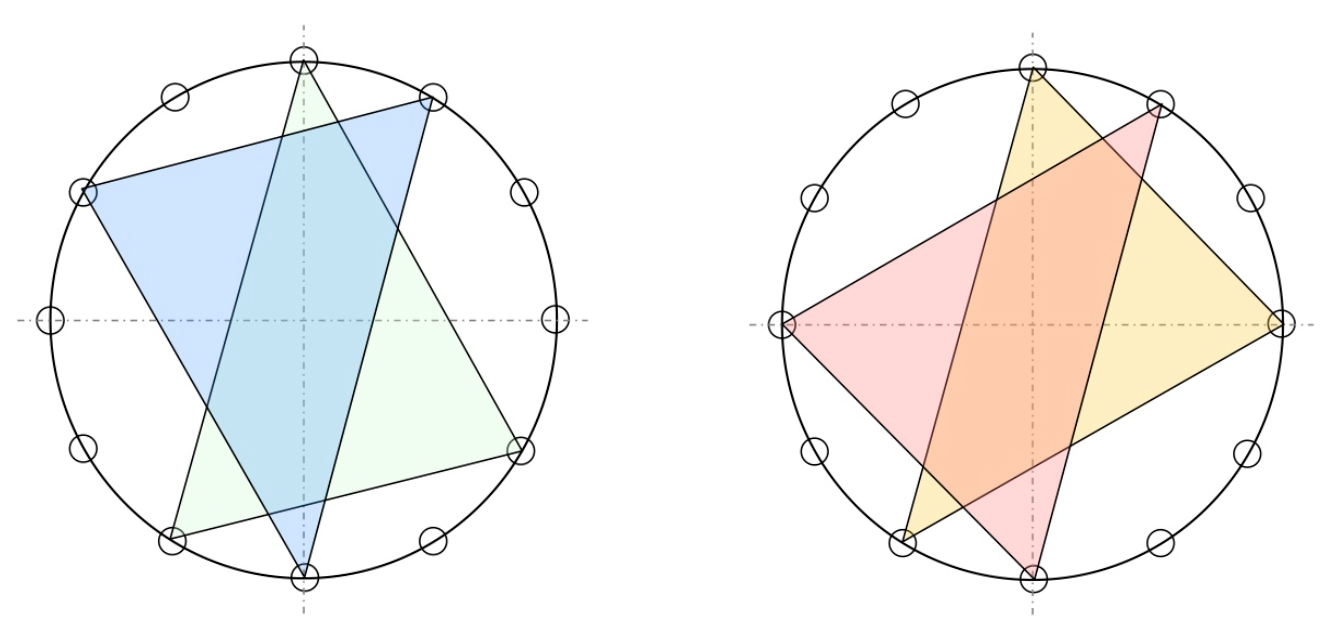(Btw. if you are interested in the full musical story, I strongly recommend the alpof blog by Alexandre Popoff, from which the above picture is taken.)

Clearly, there are $12$ major triads (one for each root), and $12$ minor triads.

From the shape of the triad-triangles it is also clear that rotations (transpositions) send major triads to major triads (and minors to minors), and that reflexions (involutions) interchange major with minor triads.

That is, the dihedral group $D_{12}$ (or if you prefer the $T/I$-group) acts on the set of $24$ major and minor triads, and this action is transitive (an element stabilising a triad-triangle must preserve its type (so is a rotation) and its root (so must be the identity)).

Can we hear the action of the very special group element $T_6$ (the unique non-trivial central element of $D_{12}$) on the chords?

This action is not only the transposition by three full tones, but also a point-reflexion with respect to the center of the $12$-gon (see two examples in the picture above). This point reflexion can be compositionally meaningful to refer to two very different upside-down worlds.

In It’s $T_6$-day, Alexandre Popoff gives several examples. Here’s one of them, the Ark theme in Indiana Jones – Raiders of the Lost Ark.

“The $T_6$ transformation is heard throughout the map room scene (in particular at 2:47 in the video): that the ark is a dreadful object from a very different world is well rendered by the $T_6$ transposition, with its inherent tritone and point reflection.”

Let’s move on in the direction of the Elephant.

We saw that the only affine map of the form $x \mapsto \pm x + k$ fixing say the major $0$-triad $(0,4,7)$ is the identity map.

But, we can ask for the collection of all affine maps $x \mapsto a x + b$ fixing this major $0$-triad set-wise, that is, such that
$\{ b, 4a+b~\text{mod}~12, 7a+b~\text{mod}~2 \} \subseteq \{ 0,4,7 \}$

A quick case-by-case analysis shows that there are just eight such maps: the identity and the constant maps
$x \mapsto x,~x \mapsto 0,~x \mapsto 4, ~x \mapsto 7$
and the four maps
$\underbrace{x \mapsto 3x+7}_a,~\underbrace{x \mapsto 8x+4}_b,~x \mapsto 9x+4,~x \mapsto 4x$

Compositions of such maps again preserve the set $\{ 0,4,7 \}$ so they form a monoid, and a quick inspection with GAP learns that $a$ and $b$ generate this monoid.

 gap> a:=Transformation([10,1,4,7,10,1,4,7,10,1,4,7]);; gap> b:=Transformation([12,8,4,12,8,4,12,8,4,12,8,4]);; gap> gens:=[a,b];; gap> T:=Monoid(gens); gap> Size(T); 8 

The monoid $T$ is the triadic monoid of Thomas Noll’s paper The topos of triads.

The monoid $T$ can be seen as a one-object category (with endomorphisms the elements of $T$). The corresponding presheaf topos is then the category of all sets equipped with a right $T$-action.

Actually, Noll considers just one such presheaf (and its sub-presheaves) namely $\mathcal{F}=\mathbb{Z}_{12}$ with the action of $T$ by affine maps described before.

He is interested in the sheafifications of these presheaves with respect to Grothendieck topologies, so we have to describe those.

For any monoid category, the subobject classifier $\Omega$ is the set of all right ideals in the monoid.

Using the GAP sgpviz package we can draw its Cayley graph (red coloured vertices are idempotents in the monoid, the blue vertex is the identity map).

 gap> DrawCayleyGraph(T);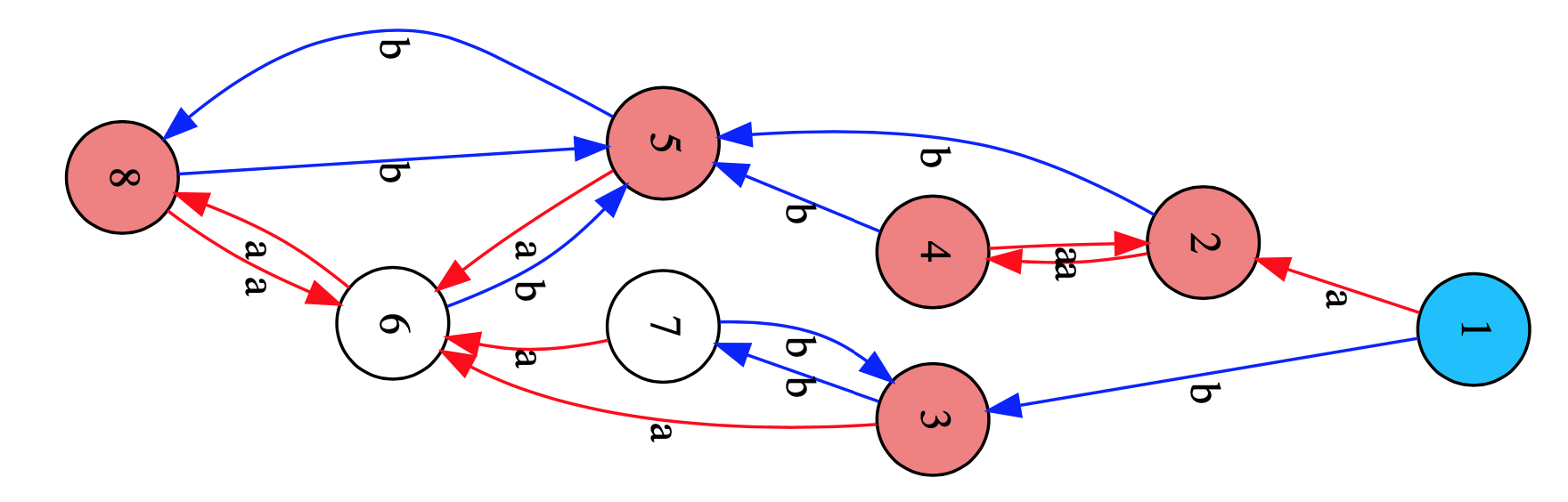The elements of $T$ (vertices) which can be connected by oriented paths (in both ways) in the Cayley graph, such as here $\{ 2,4 \}$, $\{ 3,7 \}$ and $\{ 5,6,8 \}$, will generate the same right ideal in $T$, so distinct right ideals are determined by unidirectional arrows, such as from $1$ to $2$ and $3$ or from $\{ 2,4 \}$ to $5$, or from $\{ 3,7 \}$ to $6$.

This gives us that $\Omega$ consists of the following six elements:

• $0 = \emptyset$
• $C = \{ 5,6,8 \} = a.T \wedge b.T$
• $L = \{ 2,4,5,6,8 \}=a.T$
• $R = \{ 3,7,5,6,8 \}=b.T$
• $P = \{ 2,3,4,5,6,7,8 \}=a.T \vee b.T$
• $1 = T$

As a subobject classifier $\Omega$ is itself a presheaf, so wat is the action of the triad monoid $T$ on it? For all $A \in \Omega$, and $s \in T$ the action is given by $A.s = \{ t \in T | s.t \in A \}$ and it can be read off from the Cayley-graph.

$\Omega$ is a Heyting algebra of which the inclusions, and logical operations can be summarised in the picture below, using the Hexboards and Heytings-post.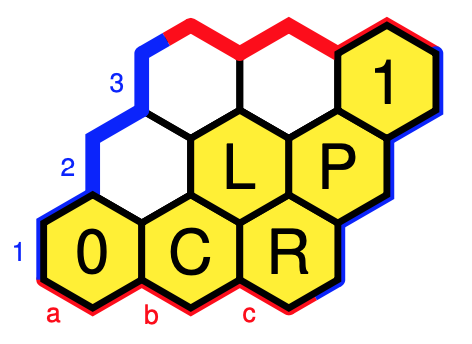In this case, Grothendieck topologies coincide with Lawvere-Tierney topologies, which come from closure operators $j~:~\Omega \rightarrow \Omega$ which are order-increasing, idempotent, and compatible with the $T$-action and with the $\wedge$, that is,

• if $A \leq B$, then $j(A) \leq j(B)$
• $j(j(A)) = j(A)$
• $j(A).t=j(A.t)$
• $j(A \wedge B) = j(A) \wedge j(B)$

Colouring all cells with the same $j$-value alike, and remaining cells $A$ with $j(A)=A$ coloured yellow, we have six such closure operations $j$, that is, Grothendieck topologies.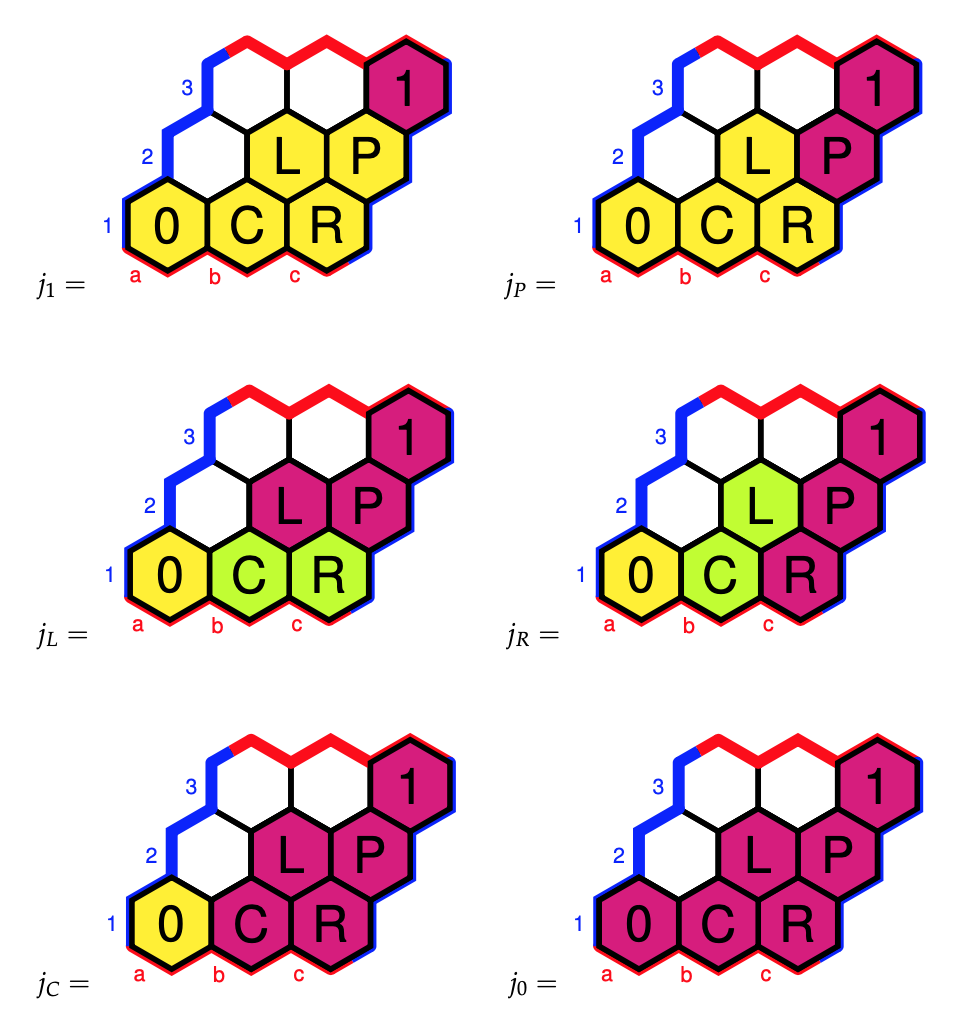The triadic monoid $T$ acts via affine transformations on the set of pitch classes $\mathbb{Z}_{12}$ and we’ve defined it such that it preserves the notes $\{ 0,4,7 \}$ of the major $(0,4,7)$-chord, that is, $\{ 0,4,7 \}$ is a subobject of $\mathbb{Z}_{12}$ in the topos of $T$-sets.

The point of the subobject classifier $\Omega$ is that morphisms to it classify subobjects, so there must be a $T$-equivariant map $\chi$ making the diagram commute (vertical arrows are the natural inclusions)
$\xymatrix{\{ 0,4,7 \} \ar[r] \ar[d] & 1 \ar[d] \\ \mathbb{Z}_{12} \ar[r]^{\chi} & \Omega}$

What does the morphism $\chi$ do on the other pitch classes? Well, it send an element $k \in \mathbb{Z}_{12} = \{ 1,2,\dots,12=0 \}$ to

• $1$ iff $k \in \{ 0,4,7 \}$
• $P$ iff $a(k)$ and $b(k)$ are in $\{ 0,4,7 \}$
• $L$ iff $a(k) \in \{ 0,4,7 \}$ but $b(k)$ is not
• $R$ iff $b(k) \in \{ 0,4,7 \}$ but $a(k)$ is not
• $C$ iff neither $a(k)$ nor $b(k)$ is in $\{ 0,4,7 \}$

Remember that $a$ and $b$ are the transformations (images of $(1,2,\dots,12)$)
 a:=Transformation([10,1,4,7,10,1,4,7,10,1,4,7]);; b:=Transformation([12,8,4,12,8,4,12,8,4,12,8,4]);; 
so we see that

• $0,1,4$ are mapped to $1$
• $3$ is mapped to $P$
• $8,11$ are mapped to $L$
• $1,6,9,10$ are mapped to $R$
• $2,5$ are mapped to $C$

Finally, we can compute the sheafification of the sub-presheaf $\{ 0,4,7 \}$ of $\mathbb{Z}$ with respect to a Grothendieck topology $j$: it consists of the set of those $k \in \mathbb{Z}_{12}$ such that $j(\chi(k)) = 1$.

The musically interesting Grothendieck topologies are $j_P, j_L$ and $j_R$ with corresponding sheaves:

• For $j_P$ we get the sheaf $\{ 0,3,4,7 \}$ which Mamuth-ers call a Major-Minor Mixture as these are the notes of both the major and minor $0$-triads
• For $j_L$ we get $\{ 0,3,4,7,8,11 \}$ which is an example of an Hexatonic scale (six notes), here they are the notes of the major and minor $0,~4$ and $8$-triads
• For $j_R$ we get $\{ 0,1,3,4,6,7,9,10 \}$ which is an example of an Octatonic scale (eight notes), here they are the notes of the major and minor $0,~3,~6$ and $9$-triads

We could have played the same game starting with the three notes of any other major triad.

Those in the know will have noticed that so far I’ve avoided another incarnation of the dihedral $D_{12}$ group in music, namely the $PLR$-group, which explains the notation for the elements of the subobject classifier $\Omega$, but this post is already way too long.

(to be continued…)

Last time I mentioned the talk “From noncommutative geometry to the tropical geometry of the scaling site” by Alain Connes, culminating in the canonical isomorphism (last slide of the talk)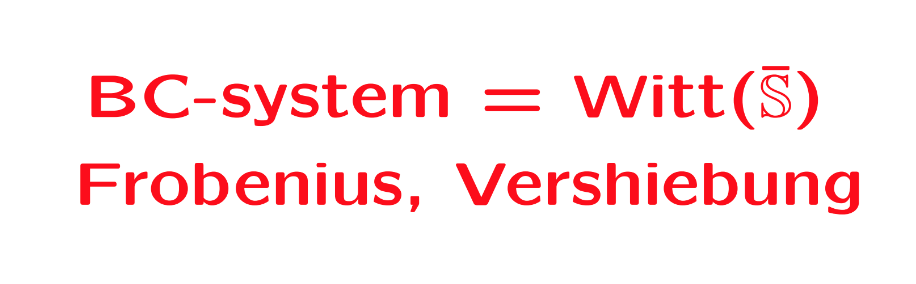Or rather, what is actually proved in his paper with Caterina Consani BC-system, absolute cyclotomy and the quantized calculus (and which they conjectured previously to be the case in Segal’s Gamma rings and universal arithmetic), is a canonical isomorphism between the $\lambda$-rings
$\mathbb{Z}[\mathbb{Q}/\mathbb{Z}] \simeq \mathbb{W}_0(\overline{\mathbb{S}})$
The left hand side is the integral groupring of the additive quotient-group $\mathbb{Q}/\mathbb{Z}$, or if you prefer, $\mathbb{Z}[\mathbf{\mu}_{\infty}]$ the integral groupring of the multiplicative group of all roots of unity $\mathbf{\mu}_{\infty}$.

The power maps on $\mathbf{\mu}_{\infty}$ equip $\mathbb{Z}[\mathbf{\mu}_{\infty}]$ with a $\lambda$-ring structure, that is, a family of commuting endomorphisms $\sigma_n$ with $\sigma_n(\zeta) = \zeta^n$ for all $\zeta \in \mathbf{\mu}_{\infty}$, and a family of linear maps $\rho_n$ induced by requiring for all $\zeta \in \mathbf{\mu}_{\infty}$ that
$\rho_n(\zeta) = \sum_{\mu^n=\zeta} \mu$
The maps $\sigma_n$ and $\rho_n$ are used to construct an integral version of the Bost-Connes algebra describing the Bost-Connes sytem, a quantum statistical dynamical system.

On the right hand side, $\mathbb{S}$ is the sphere spectrum (an object from stable homotopy theory) and $\overline{\mathbb{S}}$ its ‘algebraic closure’, that is, adding all abstract roots of unity.

The ring $\mathbb{W}_0(\overline{\mathbb{S}})$ is a generalisation to the world of spectra of the Almkvist-ring $\mathbb{W}_0(R)$ defined for any commutative ring $R$, constructed from pairs $(E,f)$ where $E$ is a projective $R$-module of finite rank and $f$ an $R$-endomorphism on it. Addition and multiplication are coming from direct sums and tensor products of such pairs, with zero element the pair $(0,0)$ and unit element the pair $(R,1_R)$. The ring $\mathbb{W}_0(R)$ is then the quotient-ring obtained by dividing out the ideal consisting of all zero-pairs $(E,0)$.

The ring $\mathbb{W}_0(R)$ becomes a $\lambda$-ring via the Frobenius endomorphisms $F_n$ sending a pair $(E,f)$ to the pair $(E,f^n)$, and we also have a collection of linear maps on $\mathbb{W}_0(R)$, the ‘Verschiebung’-maps which send a pair $(E,f)$ to the pair $(E^{\oplus n},F)$ with
$F = \begin{bmatrix} 0 & 0 & 0 & \cdots & f \\ 1 & 0 & 0 & \cdots & 0 \\ 0 & 1 & 0 & \cdots & 0 \\ \vdots & \vdots & \vdots & & \vdots \\ 0 & 0 & 0 & \cdots & 1 \end{bmatrix}$
Connes and Consani define a notion of modules and their endomorphisms for $\mathbb{S}$ and $\overline{\mathbb{S}}$, allowing them to define in a similar way the rings $\mathbb{W}_0(\mathbb{S})$ and $\mathbb{W}_0(\overline{\mathbb{S}})$, with corresponding maps $F_n$ and $V_n$. They then establish an isomorphism with $\mathbb{Z}[\mathbb{Q}/\mathbb{Z}]$ such that the maps $(F_n,V_n)$ correspond to $(\sigma_n,\rho_n)$.

But, do we really have the go to spectra to achieve this?

All this reminds me of an old idea of Yuri Manin mentioned in the introduction of his paper Cyclotomy and analytic geometry over $\mathbb{F}_1$, and later elaborated in section two of his paper with Matilde Marcolli Homotopy types and geometries below $\mathbf{Spec}(\mathbb{Z})$.

Take a manifold $M$ with a diffeomorphism $f$ and consider the corresponding discrete dynamical system by iterating the diffeomorphism. In such situations it is important to investigate the periodic orbits, or the fix-points $Fix(M,f^n)$ for all $n$. If we are in a situation that the number of fixed points is finite we can package these numbers in the Artin-Mazur zeta function
$\zeta_{AM}(M,f) = exp(\sum_{n=1}^{\infty} \frac{\# Fix(M,f^n)}{n}t^n)$
and investigate the properties of this function.

To connect this type of problem to Almkvist-like rings, Manin considers the Morse-Smale dynamical systems, a structural stable diffeomorphism $f$, having a finite number of non-wandering points on a compact manifold $M$.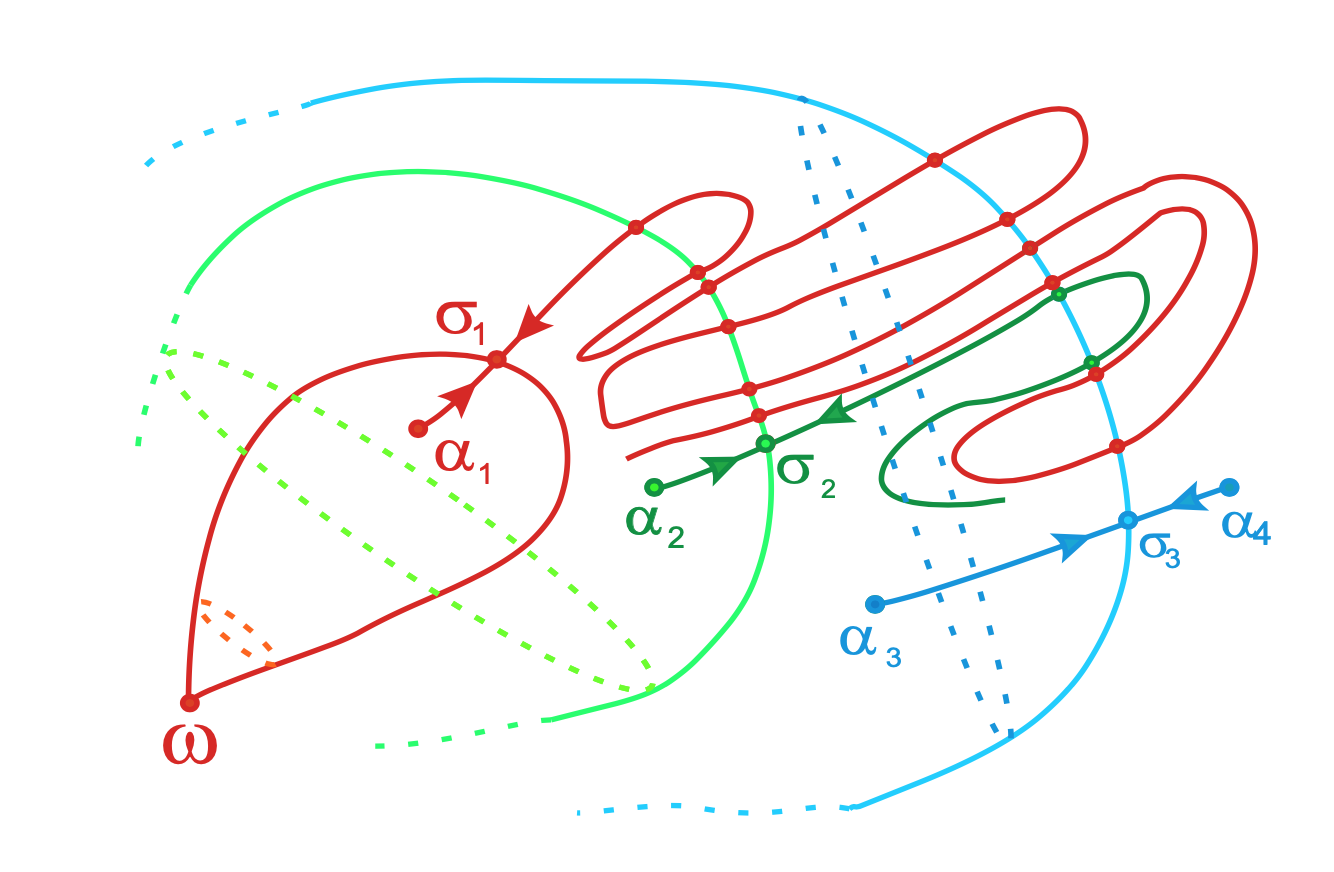From Topological classification of Morse-Smale diffeomorphisms on 3-manifolds

In such a situation $f_{\ast}$ acts on homology $H_k(M,\mathbb{Z})$, which are free $\mathbb{Z}$-modules of finite rank, as a matrix $M_f$ having only roots of unity as its eigenvalues.

Manin argues that this action is similar to the action of the Frobenius on etale cohomology groups, in which case the eigenvalues are Weil numbers. That is, one might view roots of unity as Weil numbers in characteristic one.

Clearly, all relevant data $(H_k(M,\mathbb{Z}),f_{\ast})$ belongs to the $\lambda$-subring of $\mathbb{W}_0(\mathbb{Z})$ generated by all pairs $(E,f)$ such that $M_f$ is diagonalisable and all its eigenvalues are either $0$ or roots of unity.

If we denote for any ring $R$ by $\mathbb{W}_1(R)$ this $\lambda$-subring of $\mathbb{W}_0(R)$, probably one would obtain canonical isomorphisms

– between $\mathbb{W}_1(\mathbb{Z})$ and the invariant part of the integral groupring $\mathbb{Z}[\mathbb{Q}/\mathbb{Z}]$ for the action of the group $Aut(\mathbb{Q}/\mathbb{Z}) = \widehat{\mathbb{Z}}^*$, and

– between $\mathbb{Z}[\mathbb{Q}/\mathbb{Z}]$ and $\mathbb{W}_1(\mathbb{Z}(\mathbf{\mu}_{\infty}))$ where $\mathbb{Z}(\mathbf{\mu}_{\infty})$ is the ring obtained by adjoining to $\mathbb{Z}$ all roots of unity.

Two more sources I’d like to draw from for this fall’s maths for designers-course:

A fantastic collection of handouts for a two week summer workshop entitled ’Geometry and the Imagination’, led by John Conway, Peter Doyle, Jane Gilman and Bill Thurston at the Geometry Center in Minneapolis, June 1991, based on a course ‘Geometry and the Imagination’ they taught twice before at Princeton.

Among the goodies a long list of exercises in imagining (always useful to budding architects) and how to compute curvature by peeling potatoes and other vegetables…

The course really shines in giving a unified elegant classification of the 17 wallpaper groups, the 7 frieze groups and the 14 families of spherical groups by using Thurston’s concept of orbifolds.

If you think this will be too complicated, have a look at the proof that the orbifold Euler characteristic of any symmetry pattern in the plane with bounded fundamental domain is zero :

Take a large region in the plane that is topologically a disk (i.e. without holes). Its Euler characteristic is $1$. This is approximately equal to $N$ times the orbifold Euler characteristic for some large $N$, so the orbifold Euler characteristic must be $0$.

This then leads to the Orbifold Shop where they sell orbifold parts:

• a handle for 2 Euros,
• a mirror for 1 Euro,
• a cross-cap for 1 Euro,
• an order $n$ cone point for $(n-1)/n$ Euro,
• an order $n$ corner reflector for $(n-1)/2n$ Euro, if you have the required mirrors to install this piece.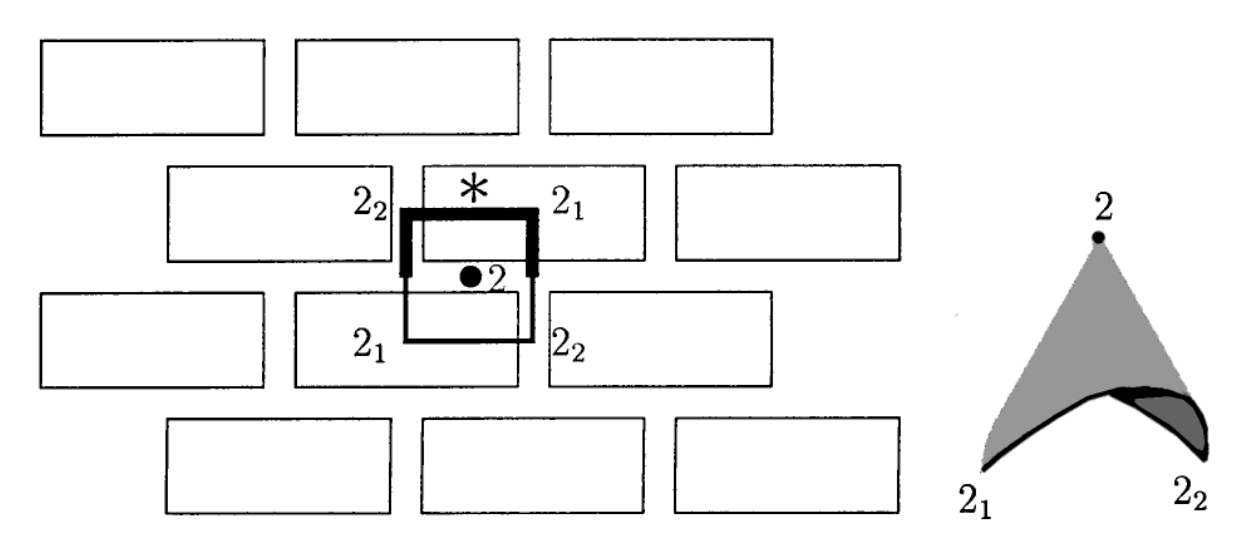Here’s a standard brick wall, with its fundamental domain and corresponding orbifold made from a mirror piece (1 Euro), two order $2$ corner reflectors (each worth $.25$ Euro), and one order $2$ cone point (worth $.5$ Euro). That is, this orbifold will cost you exactly $2$ Euros.

If you spend exactly $2$ Euros at the Orbifold Shop (and there are $17$ different ways to do this), you will have an orbifold coming from a symmetry pattern in the plane with bounded fundamental domain, that is, one of the $17$ wallpaper patterns.

For the mathematicians among you desiring more details, please read The orbifold notation for two-dimensional groups by Conway and Daniel Huson, from which the above picture was taken.

The aspiring architect should be warned that some constructions are simply not possible in 3D, even when they look convincing on paper, such as Escher’s Waterfall.M.C. Escher, Waterfall – Photo Credit

In his paper, Penrose gives a unified approach to debunk such drawings by using cohomology groups.

Clearly I have no desire to introduce cohomology, but it may still be possible to get the underlying idea across. Let’s take the Penrose triangle (all pictures below taken from Penrose’s paper)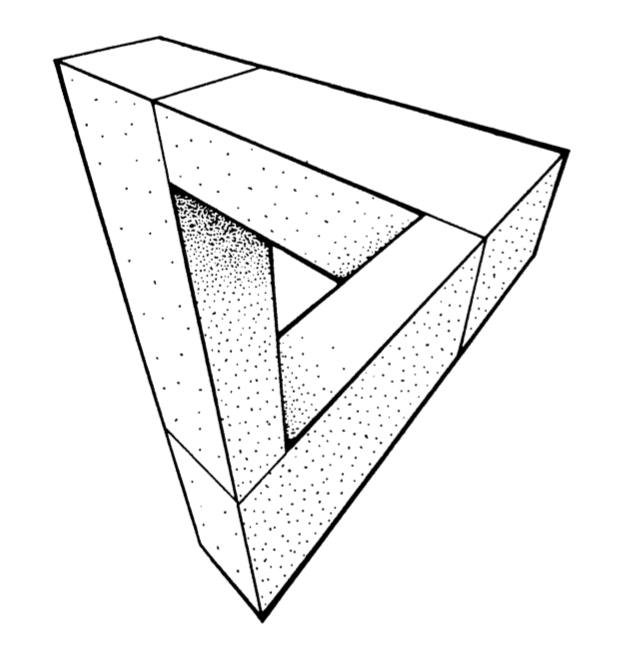The idea is to break up such a picture in several parts, each of which we do know to construct in 3D (that is, we take a particular cover of our figure). We can slice up the Penrose triangle in three parts, and if you ever played with Lego you’ll know how to construct each one of them.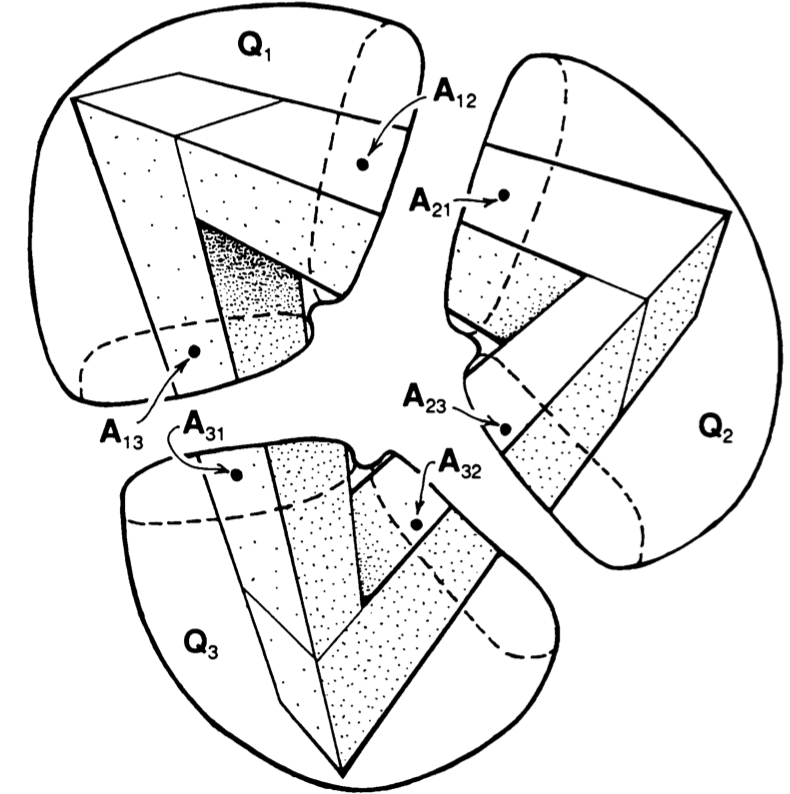Next, position the constructed pieces in space as in the picture and decide which of the two ends is closer to you. In $Q_1$ it is clear that point $A_{12}$ is closer to you than $A_{13}$, so we write $A_{12} < A_{13}$.

Similarly, looking at $Q_2$ and $Q_3$ we see that $A_{23} < A_{21}$ and that $A_{31} < A_{32}$.

Next, if we try to reassemble our figure we must glue $A_{12}$ to $A_{21}$, that is $A_{12}=A_{21}$, and similarly $A_{23}=A_{32}$ and $A_{31}=A_{13}$. But, then we get
$A_{13}=A_{31} < A_{32}=A_{23} < A_{21}=A_{12} < A_{13}$ which is clearly absurd.

Once again, if you have suggestions for more material to be included, please let me know.

In this series I’ll mention some books I found entertaining, stimulating or comforting during these Corona times. Read them at your own risk.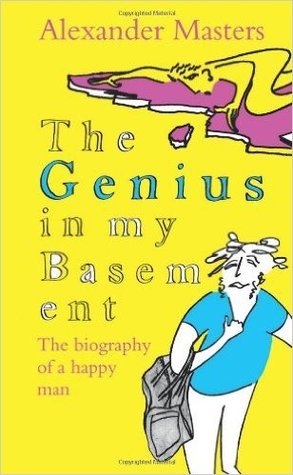This must have been the third time I’ve read The genius in by basement – The biography of a happy man by Alexander masters.

I first read it when it came out in 2011.

Then, in conjunction with Genius at play – The Curious Mind of John Horton Conway Conway’s biography by Siobhan Roberts, in july 2017, which is probably the best way to read this book.

And, then again last week, as Simon Norton‘s work pops up wherever I look, as in the previous post.

It takes some time to get used to the rather chaotic style (probably used because that’s how Masters perceives Norton), and all attempts at explaining Simon’s mathematics can better be skipped.

The book tries to find an answer as to why a child prodigy and genius like Simon Norton failed to secure a safe place in academics.

Page 328:

Simon’s second explanation of his loss of mathematical direction is heartbreaking. Now that Conway has fled to America, there is no one in the mathematical world who will work with him.

They say he is too peculiar, too shabby, too old.

His interests are fixed in mathematics that has had its day. His brilliance is frigid. His talent, perfectly suited to an extraordinary moment in algebraic history (the symmetry work at Cambridge during the early 1970s and 1980s) is out of fashion.

This may give the impression that Norton stopped doing good math after Conway left for Princeton in 1985. This is far from true.

Norton’s Wikipedia page mentions only post 1995 publications, which in itself is deplorable, as it leaves out his contributions to the ATLAS and his seminal paper with Conway on Monstrous moonshine.

Here’s Alexander Masters talking about ‘Genius in my basement’

I’ll leave you with a nice quote, comparing Monstrous Moonshine to a Sainsbury’s bag on Jupiter.

Page 334:

This much I do know: Monstrous Moonshine links the Monster to distant mathematics and the structure of space in ways that are as awe-inspiring to a man like Simon as it would be to an astronaut to step out of his space machine on Jupiter, and find a Sainsbury’s bag floating past. That’s why it’s called ‘Moonshine’, because mathematicians can even now hardly believe it.

‘I think’, said Simon, standing up from his berth and shaking crumbs and clotted blobs of oil and fish off his T-shirt onto the covers, ‘I can explain to you what Moonshine is in one sentence.’

When he really tries, Simon can be a model of clarity.

‘It is,’ he said, ‘the voice of God.’

Ps, wrt. SNORT.

An integral $n$-dimensional lattice $L$ is the set of all integral linear combinations
$L = \mathbb{Z} \lambda_1 \oplus \dots \oplus \mathbb{Z} \lambda_n$
of base vectors $\{ \lambda_1,\dots,\lambda_n \}$ of $\mathbb{R}^n$, equipped with the usual (positive definite) inner product, satisfying
$(\lambda, \mu ) \in \mathbb{Z} \quad \text{for all \lambda,\mu \in \mathbb{Z}.}$
But then, $L$ is contained in its dual lattice $L^* = Hom_{\mathbb{Z}}(L,\mathbb{Z})$, and if $L = L^*$ we say that $L$ is unimodular.

If all $(\lambda,\lambda) \in 2 \mathbb{Z}$, we say that $L$ is an even lattice. Even unimodular lattices (such as the $E_8$-lattice or the $24$ Niemeier lattices) are wonderful objects, but they can only live in dimensions $n$ which are multiples of $8$.

Just like the Conway group $Co_0 = .0$ is the group of rotations of the Leech lattice $\Lambda$, one might ask whether there is a very special lattice on which the Monster group $\mathbb{M}$ acts faithfully by rotations. If such a lattice exists, it must live in dimension at least $196883$.Simon Norton (1952-2019) – Photo Credit

A first hint of such a lattice is in Conway’s original paper A simple construction for the Fischer-Griess monster group (but not in the corresponding chapter 29 of SPLAG).

Conway writes that Simon Norton showed ‘by a very simple computations that does not even require knowledge of the conjugacy classes, that any $198883$-dimensional representation of the Monster must support an invariant algebra’, which, after adding an identity element $1$, we now know as the $196884$-dimensional Griess algebra.

Further, on page 529, Conway writes:

Norton has shown that the lattice $L$ spanned by vectors of the form $1,t,t \ast t’$, where $t$ and $t’$ are transposition vectors, is closed under the algebra multiplication and integral with respect to the doubled inner product $2(u,v)$. The dual quotient $L^*/L$ is cyclic of order some power of $4$, and we believe that in fact $L$ is unimodular.

Here, transposition vectors correspond to transpositions in $\mathbb{M}$, that is, elements of conjugacy class $2A$.

In his post, Adam considers the $196883$-dimensional lattice $L’ = L \cap 1^{\perp}$ (which has $\mathbb{M}$ as its rotation symmetry group), and asks for the minimal norm (squared) of a lattice point, which he believes is $448$, and for the number of minimal vectors in the lattice, which might be
$2639459181687194563957260000000 = 9723946114200918600 \times 27143910000$
the number of oriented arcs in the Monster graph.

Here, the Monster graph has as its vertices the elements of $\mathbb{M}$ in conjugacy class $2A$ (which has $9723946114200918600$ elements) and with an edge between two vertices if their product in $\mathbb{M}$ again belongs to class $2A$, so the valency of the graph must be $27143910000$, as explained in that old post the monster graph and McKay’s observation.

When I asked Adam whether he had more information about his lattice, he kindly informed me that Borcherds told him that the Norton lattice $L$ didn’t turn out to be unimodular after all, but that a unimodular lattice with monstrous symmetry had been constructed by Scott Carnahan in the paper A Self-Dual Integral Form of the Moonshine Module.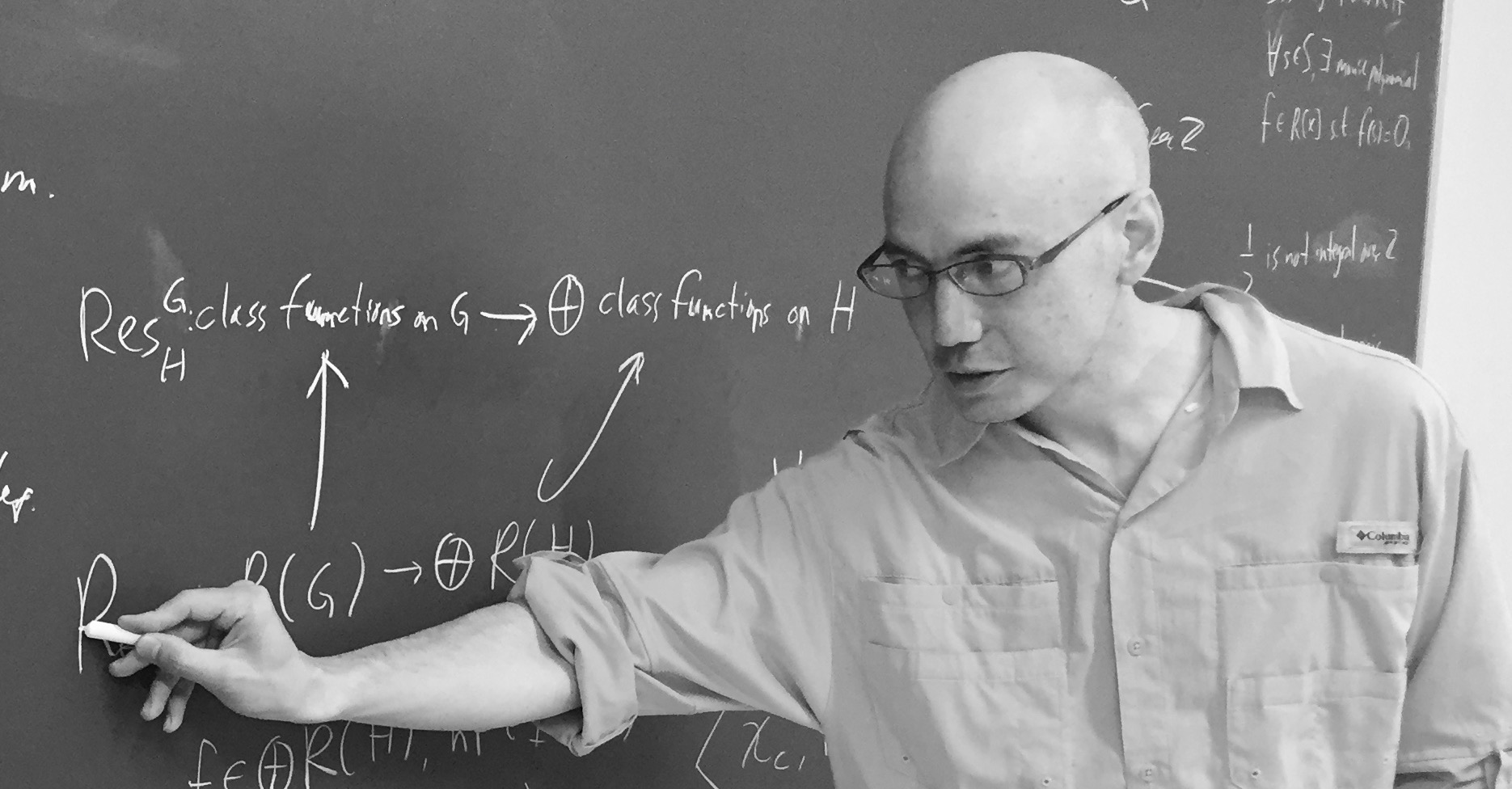Scott Carnahan – Photo Credit

The major steps (or better, the little bit of it I could grasp in this short time) in the construction of this unimodular $196884$-dimensional monstrous lattice might put a smile on your face if you are an affine scheme aficionado.

Already in his paper Vertex algebras, Kac-Moody algebras, and the Monster, Richard Borcherds described an integral form of any lattice vertex algebra. We’ll be interested in the lattice vertex algebra $V_{\Lambda}$ constructed from the Leech lattice $\Lambda$ and call its integral form $(V_{\Lambda})_{\mathbb{Z}}$.

One constructs the Moonshine module $V^{\sharp}$ from $V_{\Lambda}$ by a process called ‘cyclic orbifolding’, a generalisation of the original construction by Frenkel, Lepowsky and Meurman. In fact, there are now no less than 51 constructions of the moonshine module.

One starts with a fixed point free rotation $r_p$ of $\Lambda$ in $Co_0$ of prime order $p \in \{ 2,3,5,7,13 \}$, which one can lift to an automorphism $g_p$ of the vertex algebra $V_{\Lambda}$ of order $p$ giving an isomorphism $V_{\Lambda}/g_p \simeq V^{\sharp}$ of vertex operator algebras over $\mathbb{C}$.

For two distinct primes $p,p’ \in \{ 2,3,5,7,13 \}$ if $Co_0$ has an element of order $p.p’$ one can find one such $r_{pp’}$ such that $r_{pp’}^p=r_{p’}$ and $r_{pp’}^{p’}=r_p$, and one can lift $r_{pp’}$ to an automorphism $g_{pp’}$ of $V_{\Lambda}$ such that $V_{\Lambda}/g_{pp’} \simeq V_{\Lambda}$ as vertex operator algebras over $\mathbb{C}$.

Problem is that these lifts of automorphisms and the isomorphisms are not compatible with the integral form $(V_{\Lambda})_{\mathbb{Z}}$ of $V_{\Lambda}$, but ‘essentially’, they can be performed on
$(V_{\Lambda})_{\mathbb{Z}} \otimes_{\mathbb{Z}} \mathbb{Z}[\frac{1}{pp’},\zeta_{2pp’}]$
where $\zeta_{2pp’}$ is a primitive $2pp’$-th root of unity. These then give a $\mathbb{Z}[\tfrac{1}{pp’},\zeta_{2pp’}]$-form on $V^{\sharp}$.

Next, one uses a lot of subgroup information about $\mathbb{M}$ to prove that these $\mathbb{Z}[\tfrac{1}{pp’},\zeta_{2pp’}]$-forms of $V^{\sharp}$ have $\mathbb{M}$ as their automorphism group.

Then, using all his for different triples in $\{ 2,3,5,7,13 \}$ one can glue and use faithfully flat descent to get an integral form $V^{\sharp}_{\mathbb{Z}}$ of the moonshine module with monstrous symmetry and such that the inner product on $V^{\sharp}_{\mathbb{Z}}$ is positive definite.

Finally, one looks at the weight $2$ subspace of $V^{\sharp}_{\mathbb{Z}}$ which gives us our Carnahan’s $196884$-dimensional unimodular lattice with monstrous symmetry!

Beautiful as this is, I guess it will be a heck of a project to deduce even the simplest of facts about this wonderful lattice from running through this construction.

For example, what is the minimal length of vectors? What is the number of minimal length vectors? And so on. All info you might have is very welcome.

Two lattices $L$ and $L’$ in the same vector space are called neighbours if their intersection $L \cap L’$ is of index two in both $L$ and $L’$.

In 1957, Martin Kneser gave a method to find all unimodular lattices (of the same dimension and signature) starting from one such unimodular lattice, finding all its neighbours, and repeating this with the new lattices obtained.

In other words, Kneser’s neighbourhood graph, with vertices the unimodular lattices (of fixed dimension and signature) and edges between them whenever the lattices are neighbours, is connected.Martin Kneser (1928-2004) – Photo Credit

Last time, we’ve constructed the Niemeier lattice $(A_1^{24})^+$ from the binary Golay code $\mathcal{C}_{24}$
$L = (A_1^{24})^+ = \mathcal{C}_{24} \underset{\mathbb{F}_2}{\times} (A_1^{24})^* = \{ \tfrac{1}{\sqrt{2}} \vec{v} ~|~\vec{v} \in \mathbb{Z}^{\oplus 24},~v=\vec{v}~mod~2 \in \mathcal{C}_{24} \}$
With hindsight, we know that $(A_1^{24})^+$ is the unique neighbour of the Leech lattice in the Kneser neighbourhood graph of the positive definite, even unimodular $24$-dimensional lattices, aka the Niemeier lattices.

Let’s try to construct the Leech lattice $\Lambda$ from $L=(A_1^{24})^+$ by Kneser’s neighbour-finding trick.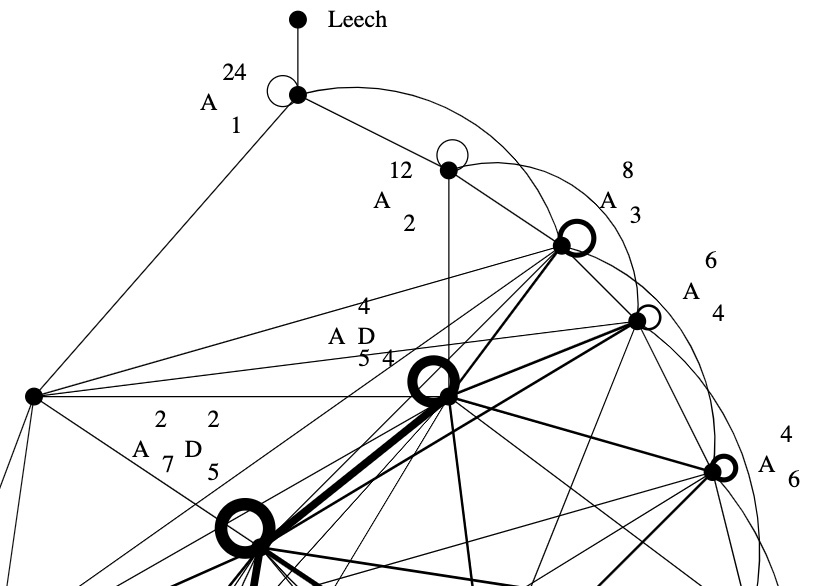Sublattices of $L$ of index two are in one-to-one correspondence with non-zero elements in $L/2L$. Take $l \in L – 2L$ and $m \in L$ such that the inner product $l.m$ is odd, then
$L_l = \{ x \in L~|~l.x~\text{is even} \}$
is an index two sublattice because $L = L_l \sqcup (L_l+m)$. By definition $l.x$ is even for all $x \in L_l$ and therefore $\frac{l}{2} \in L_l^*$. We have this situation
$L_l \subsetneq L = L^* \subsetneq L_l^*$
and $L_l^*/L_l \simeq \mathbb{F}_2 \oplus \mathbb{F}_2$, with the non-zero elements represented by $\{ \frac{l}{2}, m, \frac{l}{2}+m \}$. That is,
$L_l^* = L_l \sqcup (L_l+m) \sqcup (L_l+\frac{l}{2}) \sqcup (L_l+(\frac{l}{2}+m))$
This gives us three lattices
$\begin{cases} M_1 &= L_l \sqcup (L_l+m) = L \\ M_2 &= L_l \sqcup (L_l+\frac{l}{2}) \\ M_3 &= L_l \sqcup (L_l+(\frac{l}{2}+m)) \end{cases}$
and all three of them are unimodular because
$L_l \subsetneq M_i \subseteq M_i^* \subsetneq L_l^*$
and $L_l$ is of index $4$ in $L_l^*$.

Now, let’s assume the norm of $l$, that is, $l.l \in 4 \mathbb{Z}$. Then, either the norm of $\frac{l}{2}$ is odd (but then the norm of $\frac{l}{2}+m$ must be even), or the norm of $\frac{l}{2}$ is even, in which case the norm of $\frac{l}{2}+m$ is odd.

That is, either $M_2$ or $M_3$ is an even unimodular lattice, the other one being an odd unimodular lattice.

Let’s take for $l$ and $m$ the vectors $\lambda = \frac{1}{\sqrt{2}} (1,1,\dots,1) \in L – 2L$ and $\mu = \sqrt{2}(1,0,\dots,0) \in L$, then
$\lambda.\lambda = \frac{1}{2}\times 24 = 12 \quad \text{and} \quad \mu.\lambda = 1$
Because $\frac{\lambda}{2}.\frac{\lambda}{2} = \frac{12}{4}=3$ is odd, we have that
$\Lambda = L_{\lambda} \sqcup (L_{\lambda} + (\frac{\lambda}{2} + \mu))$
is an even unimodular lattice, which is the Leech lattice, and
$\Lambda_{odd} = L_{\lambda} \sqcup (L_{\lambda} + \frac{\lambda}{2})$
is an odd unimodular lattice, called the odd Leech lattice.John Leech (1926-1992) – Photo Credit

Let’s check that these are indeed the Leech lattices, meaning that they do not contain roots (vectors of norm two).

The only roots in $L = (A_1^{24})^+$ are the $48$ roots of $A_1^{24}$ and they are of the form $\pm \sqrt{2} [ 1, 0^{23} ]$, but none of them lies in $L_{\lambda}$ as their inproduct with $\lambda$ is one. So, all non-zero vectors in $L_{\lambda}$ have norm $\geq 4$.

As for the other part of $\Lambda$ and $\Lambda_{odd}$
$(L_{\lambda} + \frac{\lambda}{2}) \sqcup (L_{\lambda} + \mu + \frac{\lambda}{2}) = (L_{\lambda} \sqcup (L_{\lambda}+\mu))+\frac{\lambda}{2} = L + \frac{\lambda}{2}$
From the description of $L=(A_1^{24})^+$ it follows that every coordinate of a vector in $L + \frac{\lambda}{2}$ is of the form
$\frac{1}{\sqrt{2}}(v+\frac{1}{2}) \quad \text{or} \quad \frac{1}{\sqrt{2}}(v+\frac{3}{2})$
with $v \in 2 \mathbb{Z}$, with the second case instances forming a codeword in $\mathcal{C}_{24}$. In either case, the square of each of the $24$ coordinates is $\geq \frac{1}{8}$, so the norm of such a vector must be $\geq 3$, showing that there are no roots in this region either.

If one takes for $l$ a vector of the form $\frac{1}{\sqrt{2}} v = \frac{1}{\sqrt{2}}[1^a,0^{24-a}]$ where $a=8,12$ or $16$ and $v \in \mathcal{C}_{24}$, takes $m=\mu$ as before, and repeats the construction, one gets the other Niemeier-neighbours of $(A_1^{24})^+$, that is, the lattices $(A_2^{12})^+$, $(A_3^8)^+$ and $(D_4^6)^+$.

For $a=12$ one needs a slightly different argument, see section 0.2 of Richard Borcherds’ Ph.D. thesis.

Here’s the upper part of Kneser‘s neighbourhood graph of the Niemeier lattices: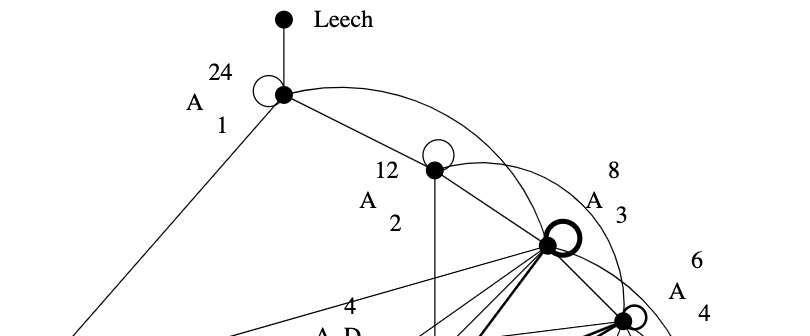The Leech lattice has a unique neighbour, that is, among the $23$ remaining Niemeier lattices there is a unique one, $(A_1^{24})^+$, sharing an index two sub-lattice with the Leech.

How would you try to construct $(A_1^{24})^+$, an even unimodular lattice having the same roots as $A_1^{24}$?

The root lattice $A_1$ is $\sqrt{2} \mathbb{Z}$. It has two roots $\pm \sqrt{2}$, determinant $2$, its dual lattice is $A_1^* = \tfrac{1}{\sqrt{2}} \mathbb{Z}$ and we have $A_1^*/A_1 \simeq C_2 \simeq \mathbb{F}_2$.

Thus, $A_1^{24}= \sqrt{2} \mathbb{Z}^{\oplus 24}$ has $48$ roots, determinant $2^{24}$, its dual lattice is $(A_1^{24})^* = \tfrac{1}{\sqrt{2}} \mathbb{Z}^{\oplus 24}$ and the quotient group $(A_1^{24})^*/A_1^{24}$ is $C_2^{24}$ isomorphic to the additive subgroup of $\mathbb{F}_2^{\oplus 24}$.

A larger lattice $A_1^{24} \subseteq L$ of index $k$ gives for the dual lattices an extension $L^* \subseteq (A_1^{24})^*$, also of index $k$. If $L$ were unimodular, then the index has to be $2^{12}$ because we have the situation
$A_1^{24} \subseteq L = L^* \subseteq (A_1^{24})^*$
So, Kneser’s glue vectors form a $12$-dimensional subspace $\mathcal{C}$ in $\mathbb{F}_2^{\oplus 24}$, that is,
$L = \mathcal{C} \underset{\mathbb{F}_2}{\times} (A_1^{24})^* = \{ \tfrac{1}{\sqrt{2}} \vec{v} ~|~\vec{v} \in \mathbb{Z}^{\oplus 24},~v=\vec{v}~mod~2 \in \mathcal{C} \}$
Because $L = L^*$, the linear code $\mathcal{C}$ must be self-dual meaning that $v.w = 0$ (in $\mathbb{F}_2$) for all $v,w \in \mathcal{C}$. Further, we want that the roots of $A_1^{24}$ and $L$ are the same, so the minimal number of non-zero coordinates in $v \in \mathcal{C}$ must be $8$.

That is, $\mathcal{C}$ must be a self-dual binary code of length $24$ with Hamming distance $8$.Marcel Golay (1902-1989) – Photo Credit

We now know that there is a unique such code, the (extended) binary Golay code, $\mathcal{C}_{24}$, which has

• one vector of weight $0$
• $759$ vectors of weight $8$ (called ‘octads’)
• $2576$ vectors of weight $12$ (called ‘dodecads’)
• $759$ vectors of weight $16$
• one vector of weight $24$

The $759$ octads form a Steiner system $S(5,8,24)$ (that is, for any $5$-subset $S$ of the $24$-coordinates there is a unique octad having its non-zero coordinates containing $S$).

Witt constructed a Steiner system $S(5,8,24)$ in his 1938 paper “Die $5$-fach transitiven Gruppen von Mathieu”, so it is not unthinkable that he checked the subspace of $\mathbb{F}_2^{\oplus 24}$ spanned by his $759$ octads to be $12$-dimensional and self-dual, thereby constructing the Niemeier-lattice $(A_1^{24})^+$ on that sunday in 1940.

John Conway classified all nine self-dual codes of length $24$ in which the weight
of every codeword is a multiple of $4$. Each one of these codes $\mathcal{C}$ gives a Niemeier lattice $\mathcal{C} \underset{\mathbb{F}_2}{\times} (A_1^{24})^*$, all but one of them having more roots than $A_1^{24}$.

Vera Pless and Neil Sloan classified all $26$ binary self-dual codes of length $24$.